# C语言小游戏之扫雷（万字详解）

hello，大家好，今天我们继续为大家带来一个小游戏，扫雷。相信这个游戏又是很多人的童年，那么我们今天就来实现一下这个扫雷游戏。

migration

二、游戏的基本设计

1.游戏基本思路

2.游戏基本框架

3.如何设计布置雷与排查雷？

CSDN开发云

4.数组的大小如何设置

5.数组的定义代码展示

tolua

三、游戏具体功能详解

1.初始化数组（InitBoard)

TCP与UDP

(1)函数的声明和定义

（2）初始化数组代码展示

（3）初始化数组代码如下

2.打印数组（DisplayBoard）

C认证

（1）打印数组的定义和声明

SSO

（2）代码的实现

uni-app H5+

（3）测试代码

（4）更进一步优化

os

（5）运行测试

（6）更进一步的优化界面

（7）代码实现如下

3.布置雷(SetMine)

(1)布置雷函数的定义和声明

bean标签的使用

（3）具体的布置雷实现

Java新手必做题目

（4）测试布雷

（5）布置雷的代码实现

4.排查雷（FindMine)

(1)排查雷函数的声明和定义

（2）排查雷的实现逻辑

（3)测试

（4）排雷的结束条件

（5）优化多次排查同一个位置的bug

（6）排查雷代码实现如下

test.c文件

game.h文件

game.c文件

# 一、游戏简介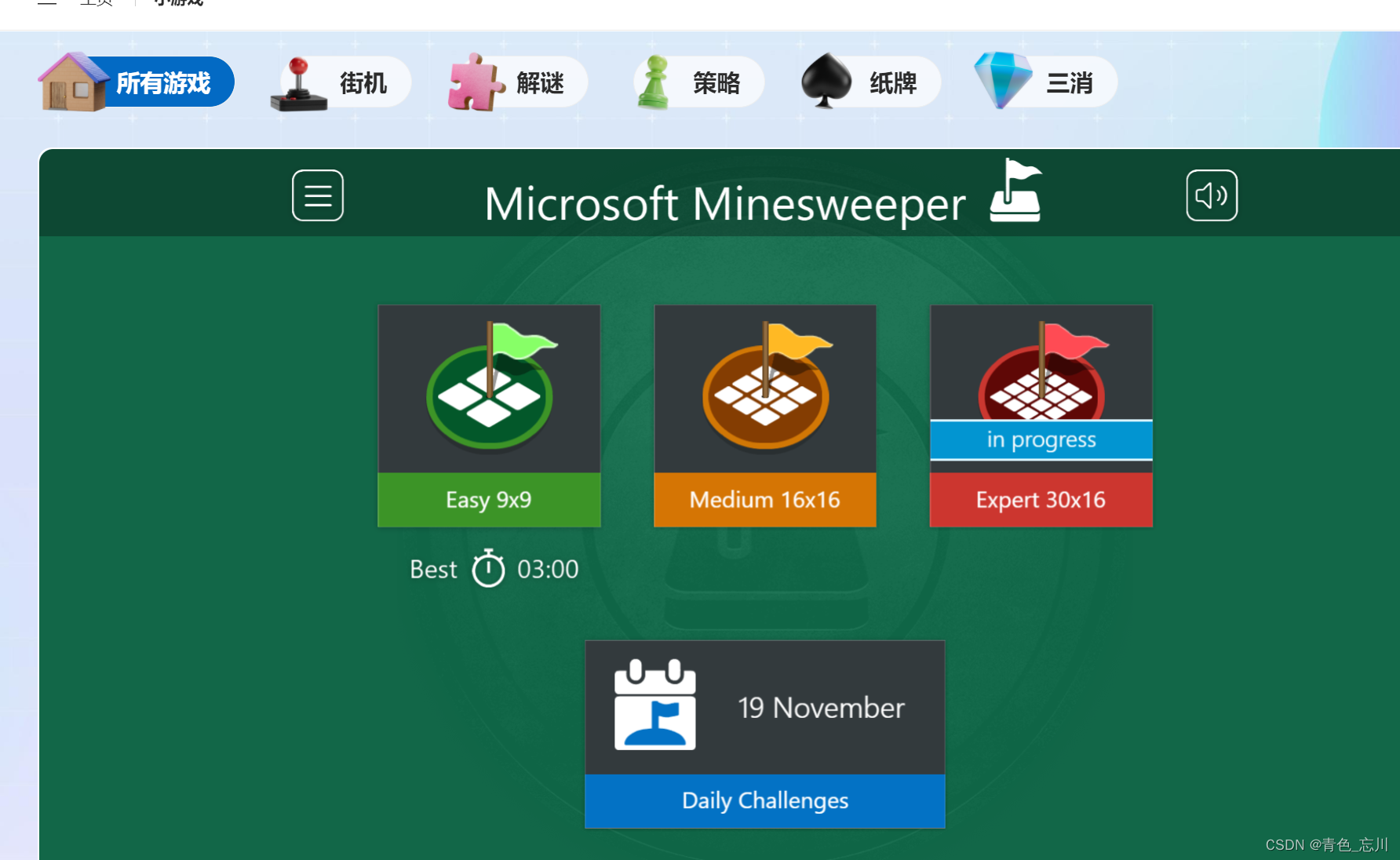点击9*9棋盘，如下图所示，每一行是9个元素，每一列也是9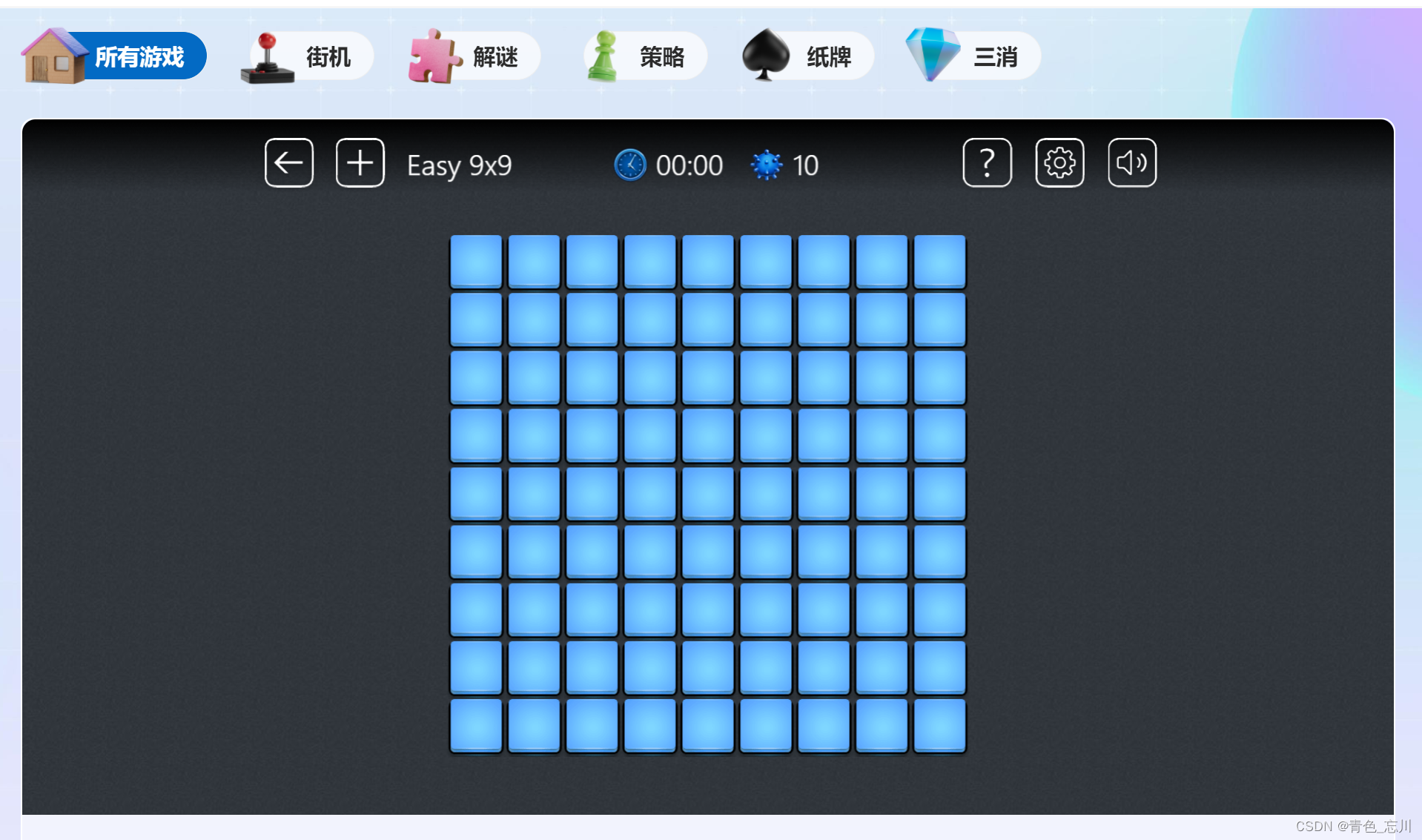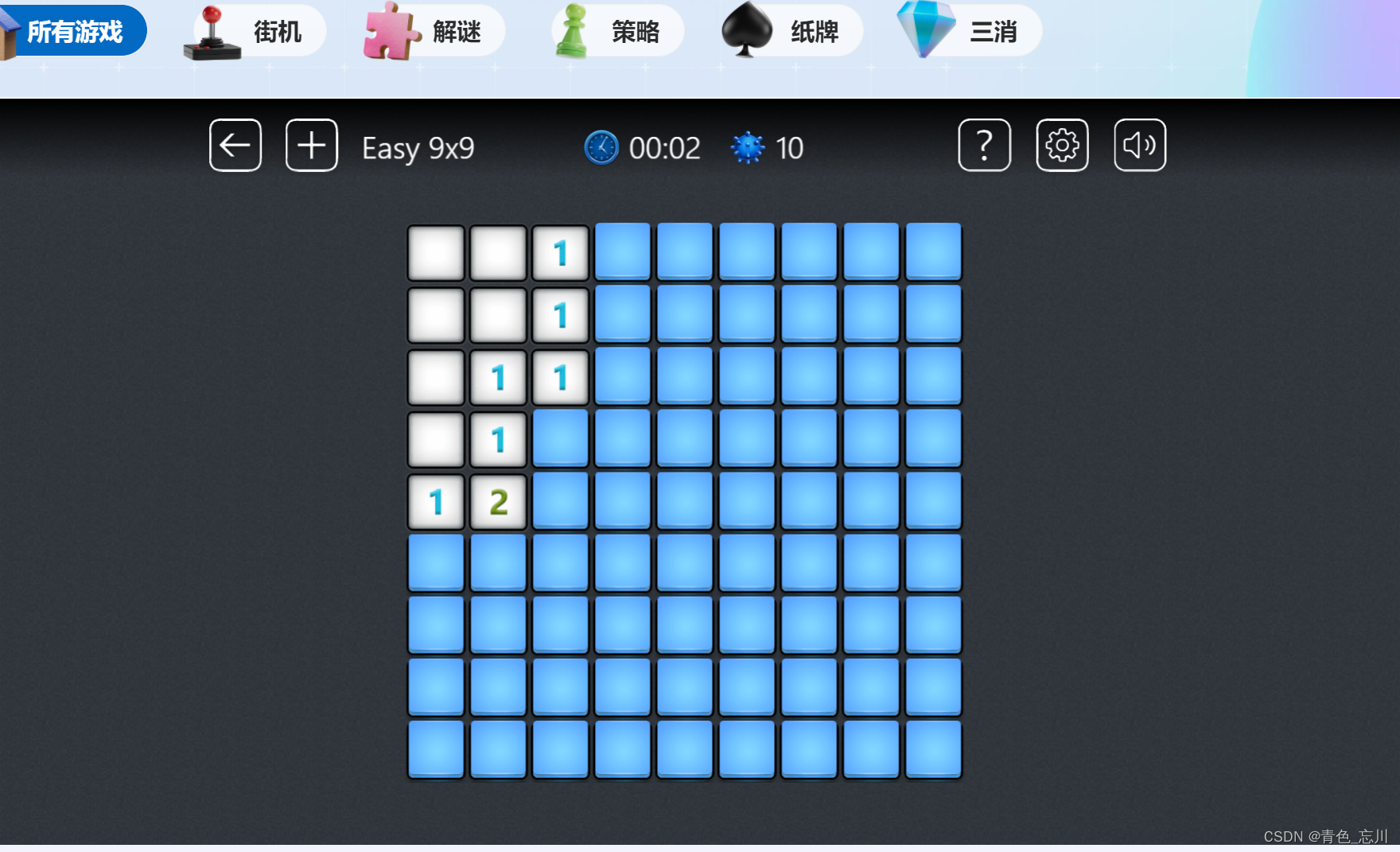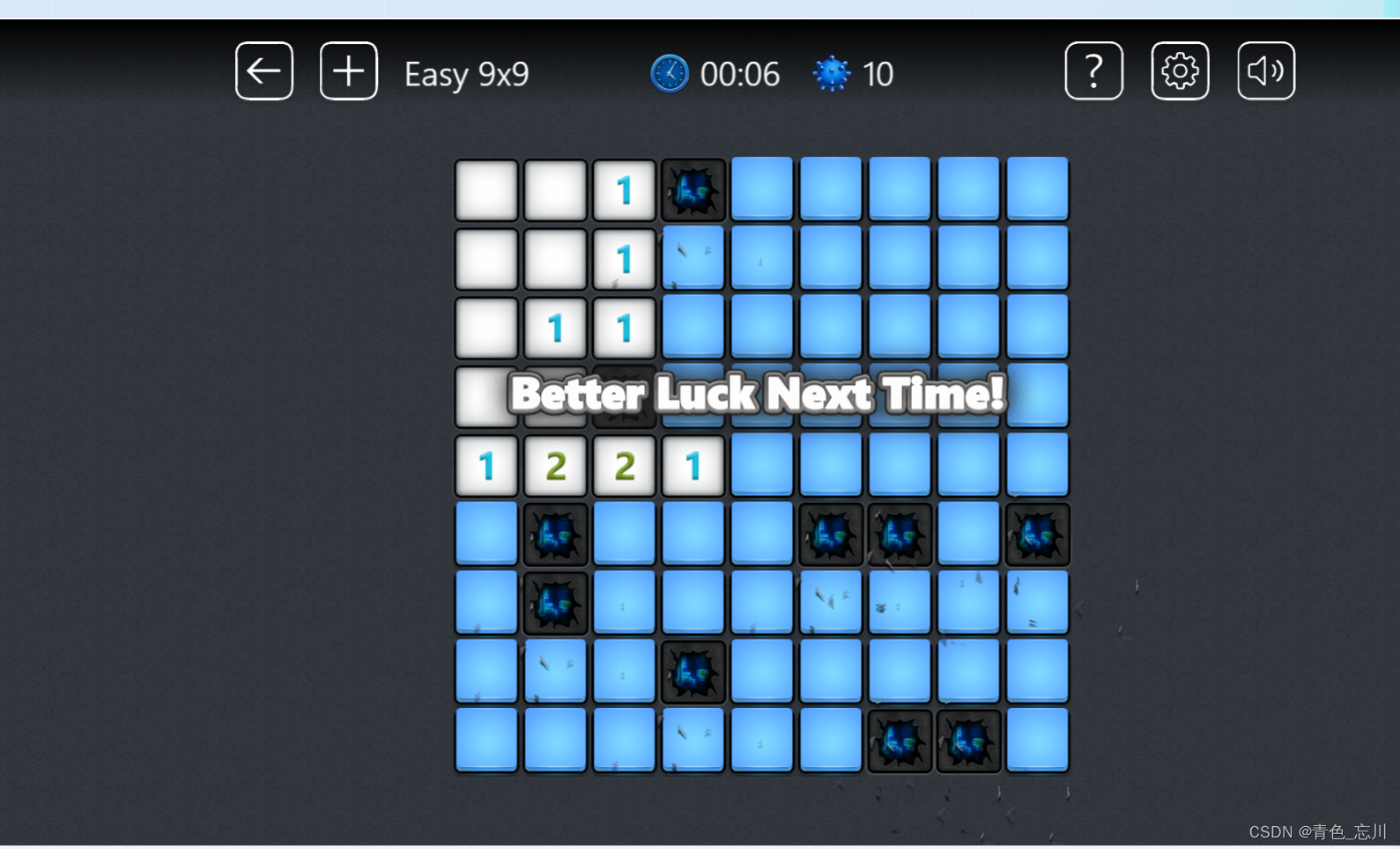# 二、游戏的基本设计

## 1.游戏基本思路

1.布置雷：创建一个二维数组，随机生成十个坐标放到位置上

2.排查雷：选择一个坐标，判断是否是雷，如果是，则爆炸，如果不是则遍历以他为中心的9个元素，并显示一个周围雷的个数。

## 2.游戏基本框架

``````#define _CRT_SECURE_NO_WARNINGS 1
#include<stdio.h>
void meau()
{
printf("*************************\n");
printf("******* 1. 开始扫雷 ******\n");
printf("******* 0. 退出游戏 ******\n");
printf("*************************\n");

}
void game()
{
printf("开始扫雷\n");
}
void test()
{
meau();
int input = 0;
do
{
printf("请输入选项>:\n");
scanf("%d", &input);
switch (input)
{
case 1:
game();
break;
case 0:
printf("退出游戏\n");
break;
default:
printf("输入错误，请重新输入\n");
break;
}
} while (input);
}
int main()
{
test();
return 0;
}``````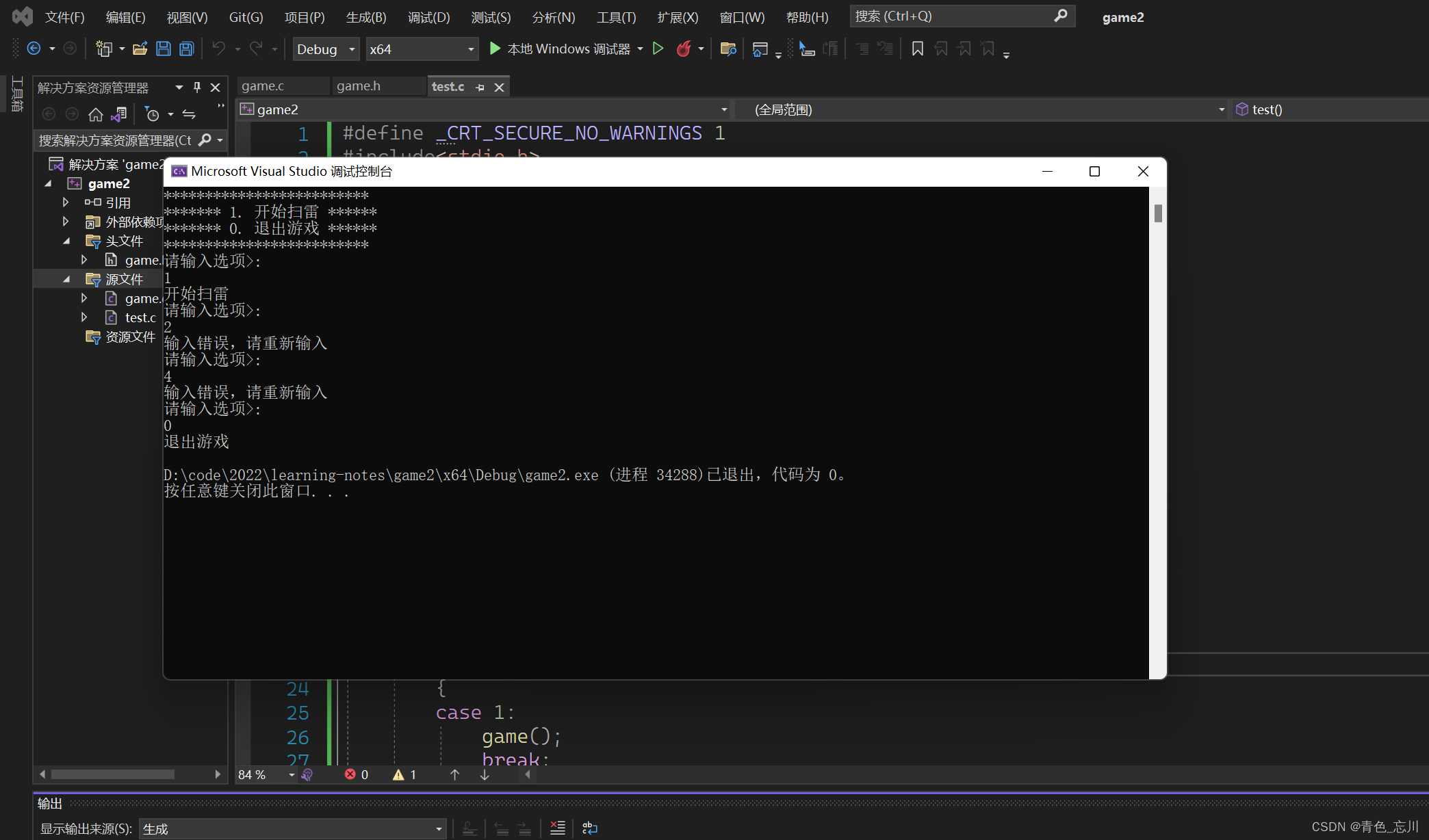## 3.如何设计布置雷与排查雷？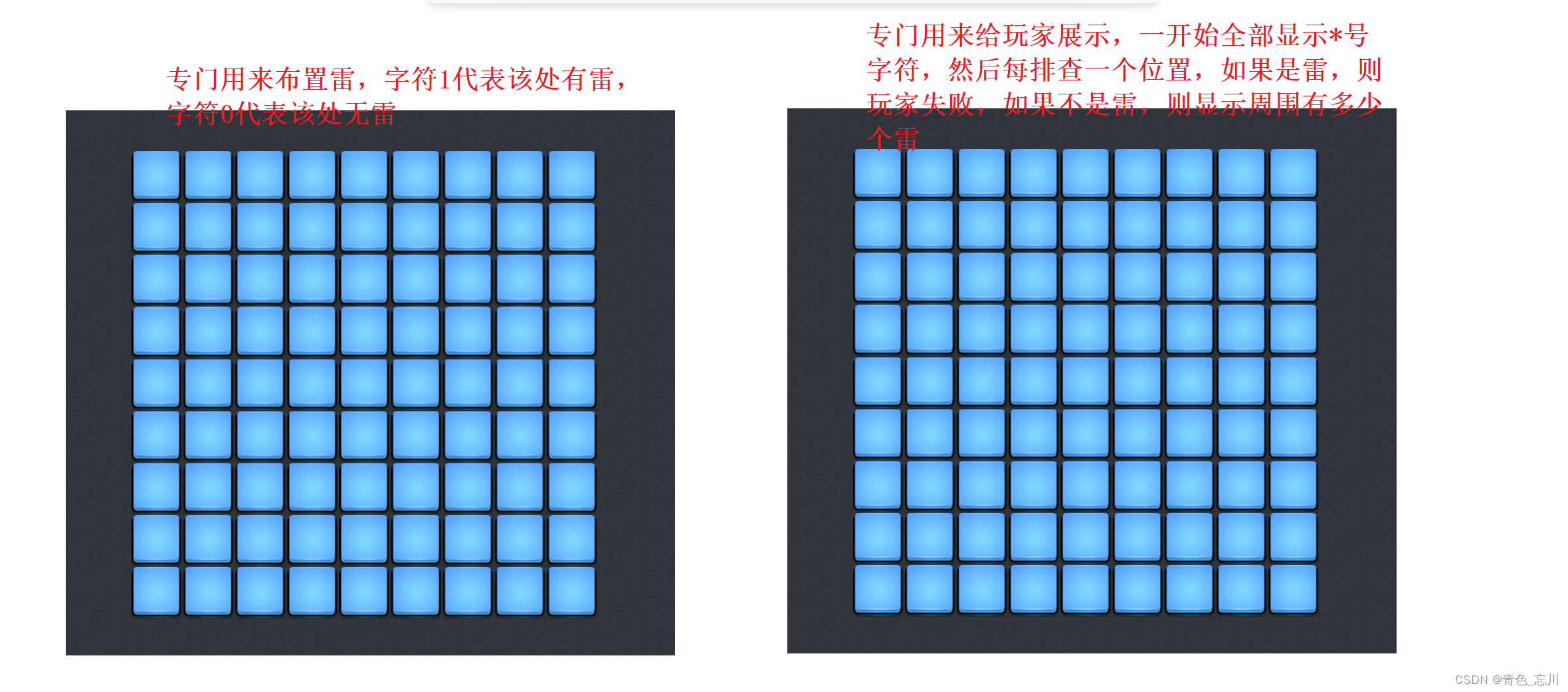## 4.数组的大小如何设置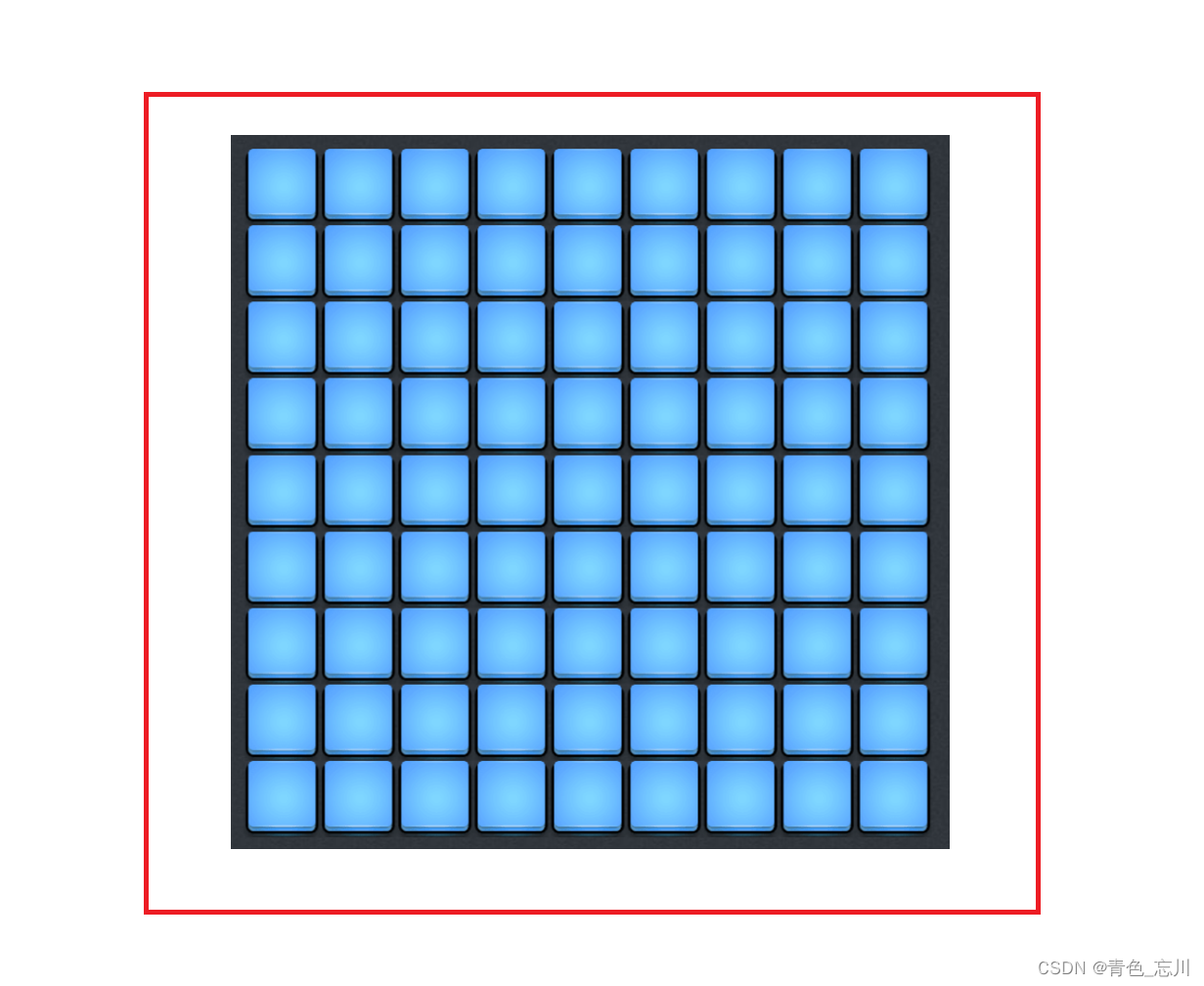## 5.数组的定义代码展示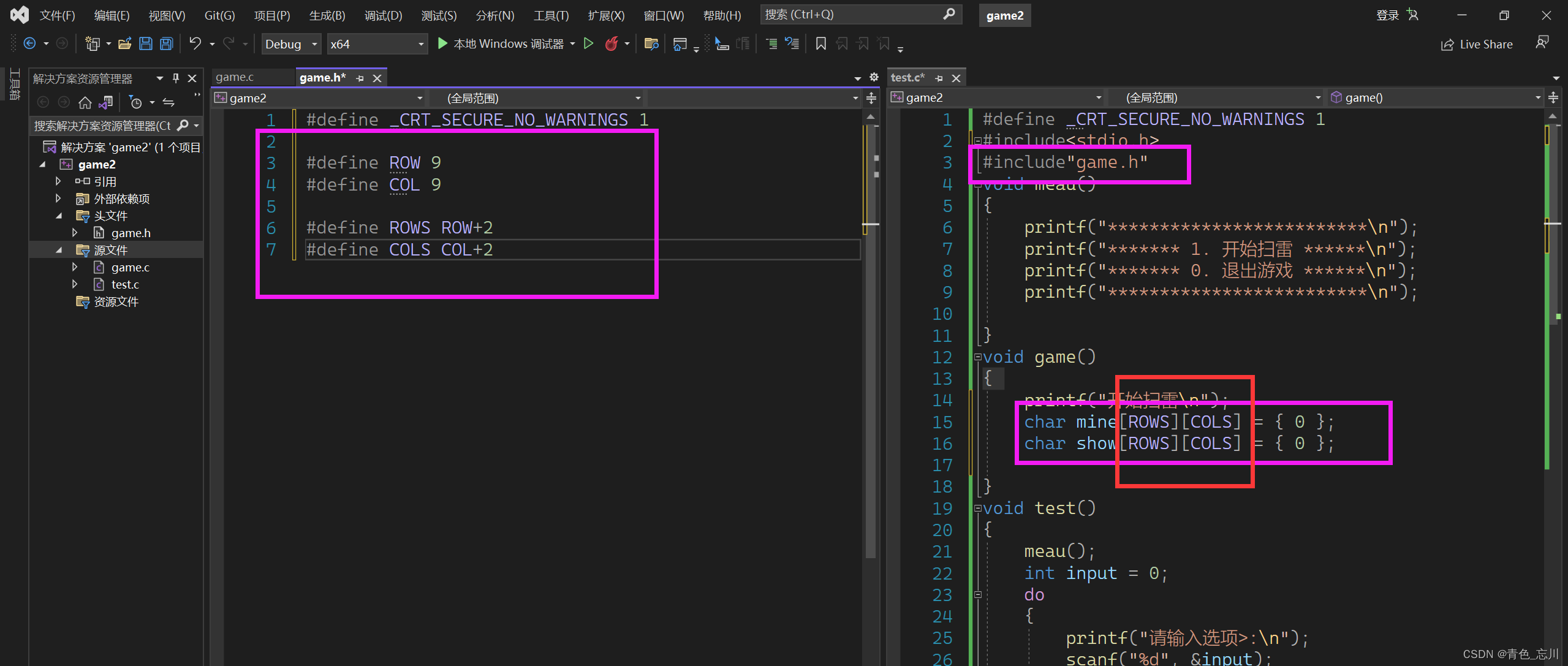# 三、游戏具体功能详解

## 1.初始化数组（InitBoard)

### (1)函数的声明和定义

``````//初始化数组
void InitBoard(char board[ROWS][COLS], int rows, int cols, char set);``````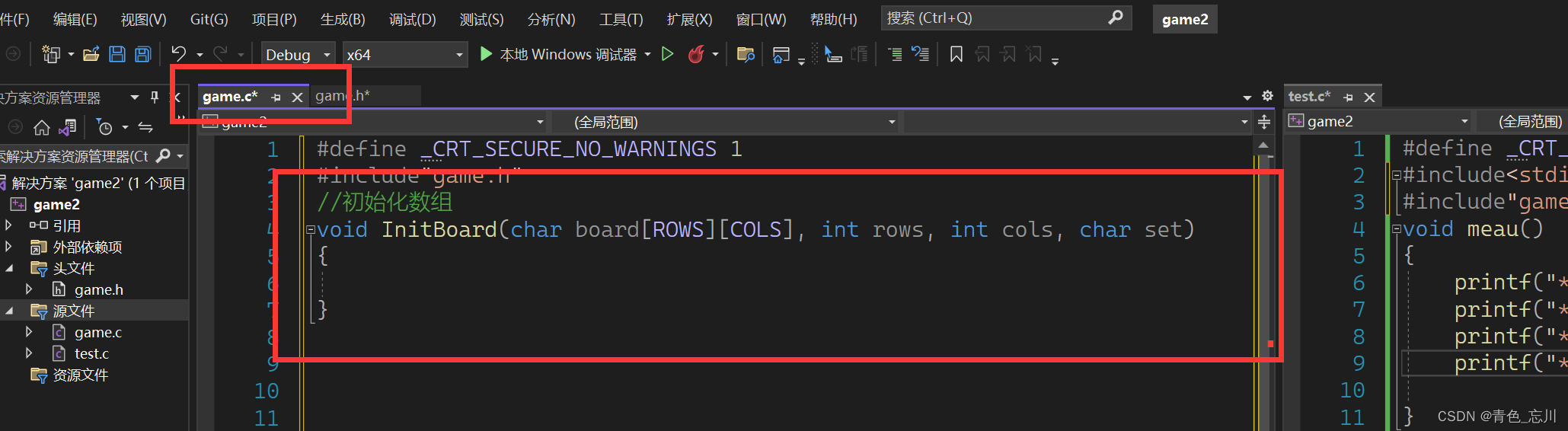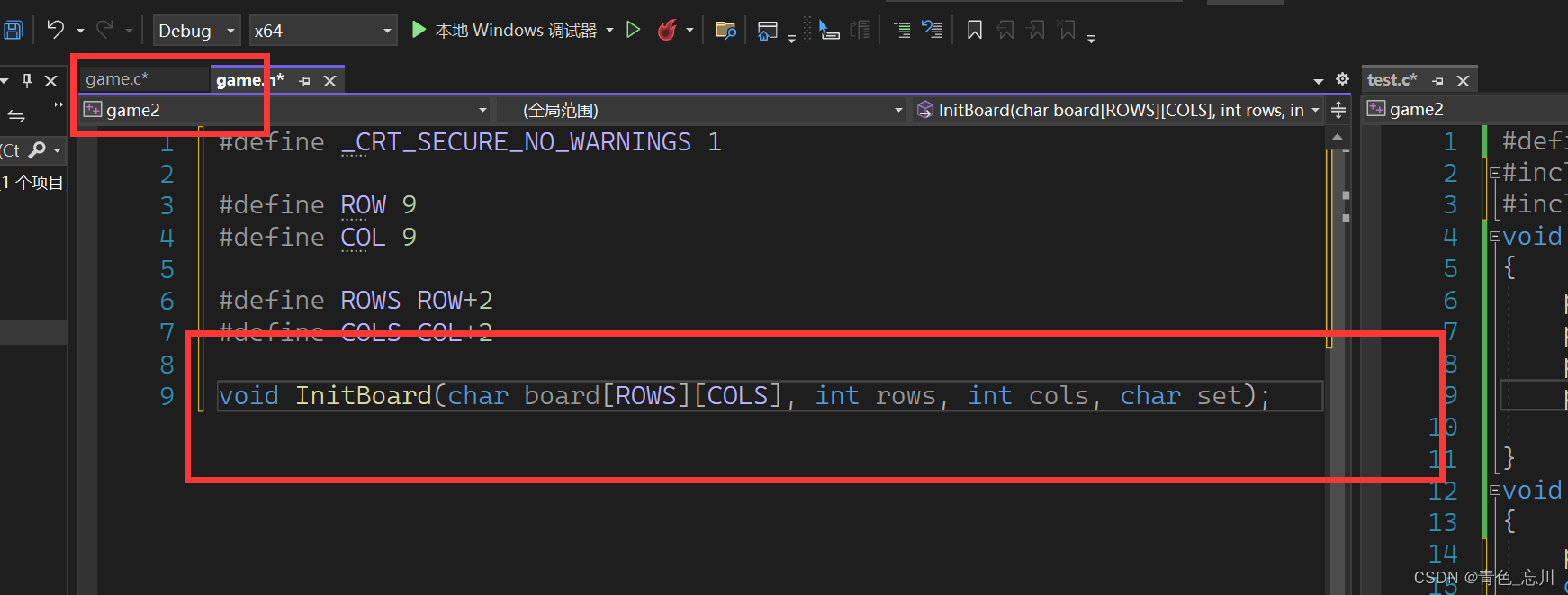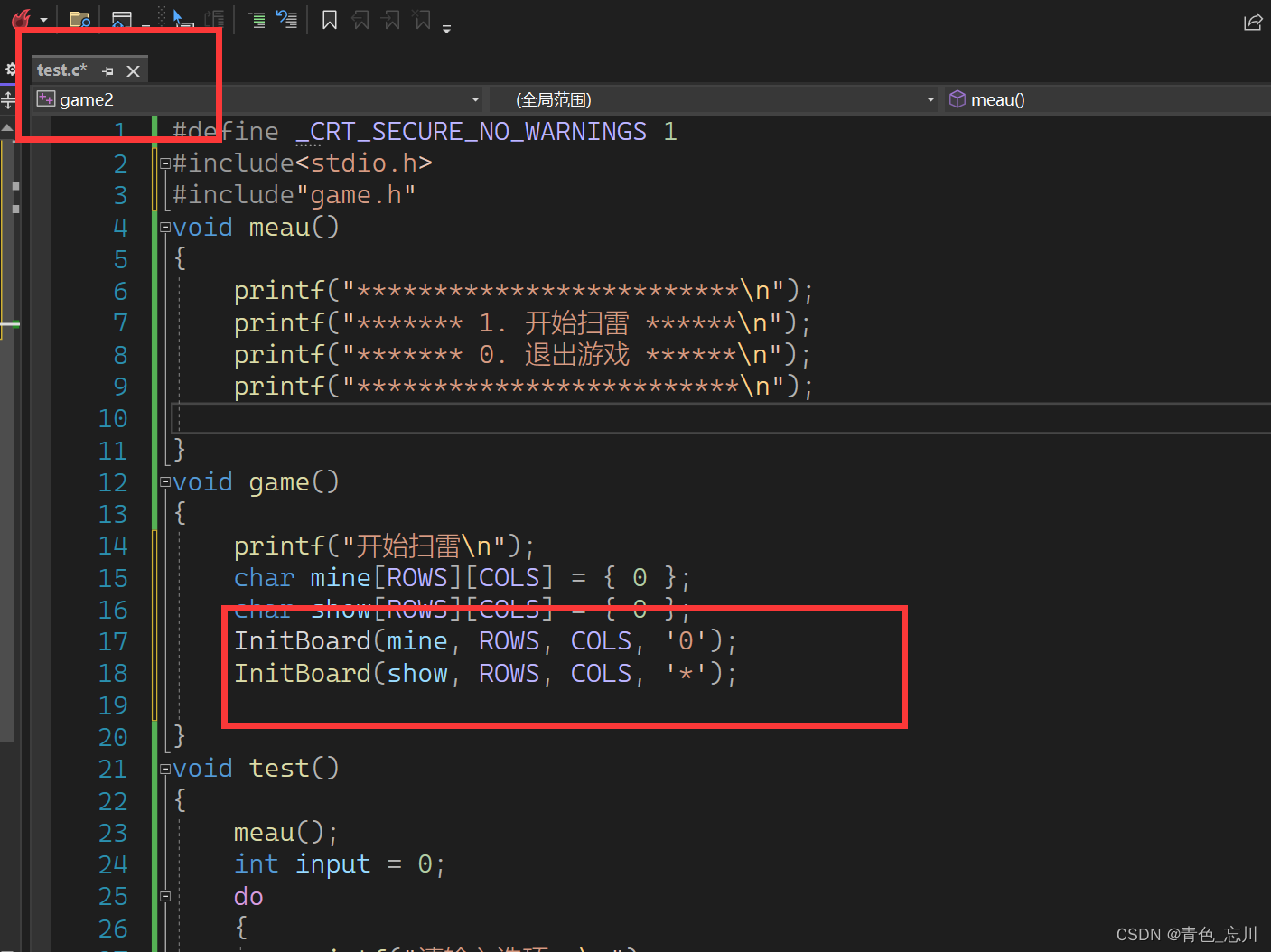### （2）初始化数组代码展示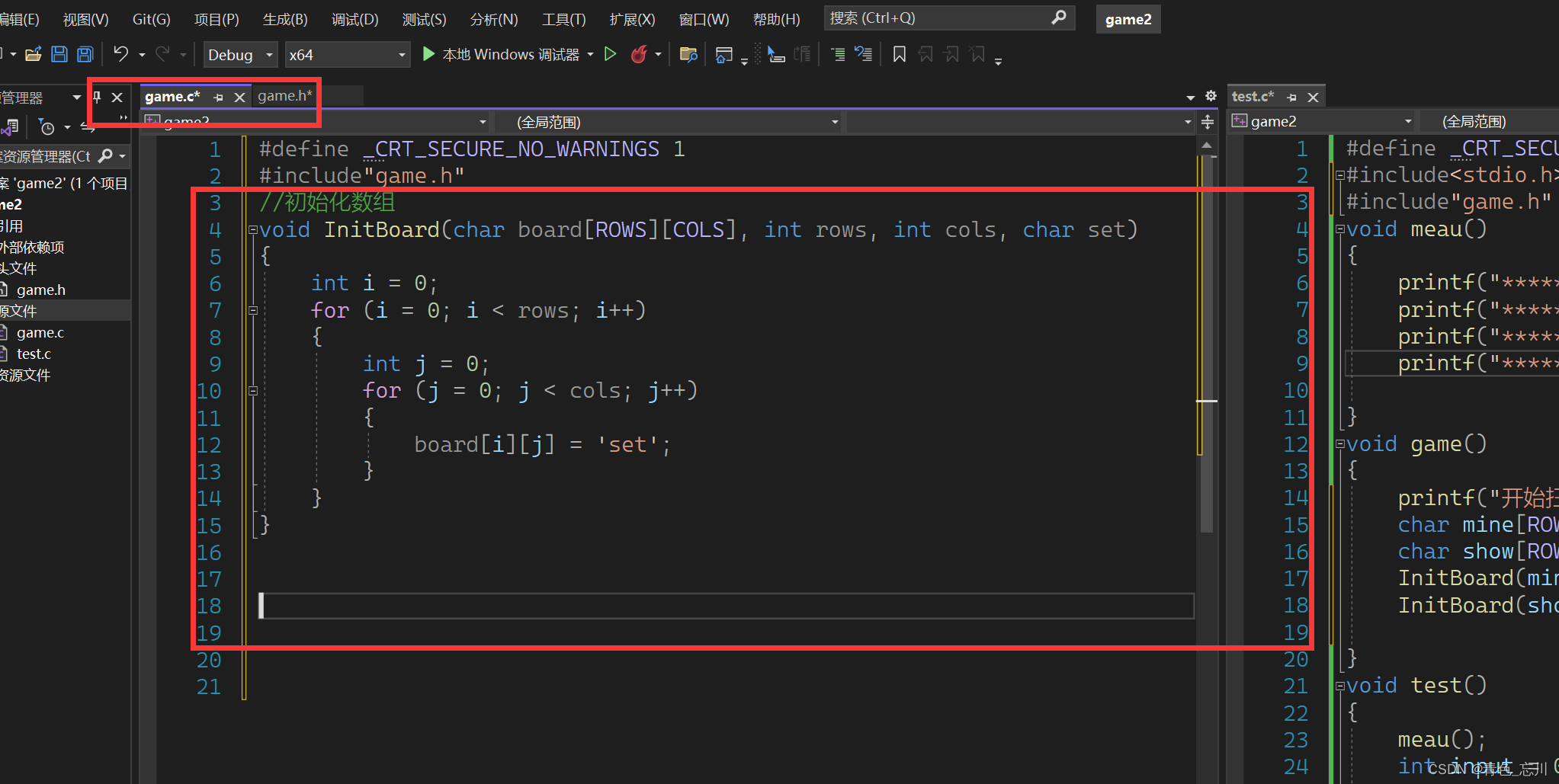### （3）初始化数组代码如下

``````//初始化数组
void InitBoard(char board[ROWS][COLS], int rows, int cols, char set)
{
int i = 0;
for (i = 0; i < rows; i++)
{
int j = 0;
for (j = 0; j < cols; j++)
{
board[i][j] = set;
}
}
}
``````

## 2.打印数组（DisplayBoard）

### （1）打印数组的定义和声明

``````//打印数组
void DisplayBoard(char board[ROWS][COLS], int row, int col);``````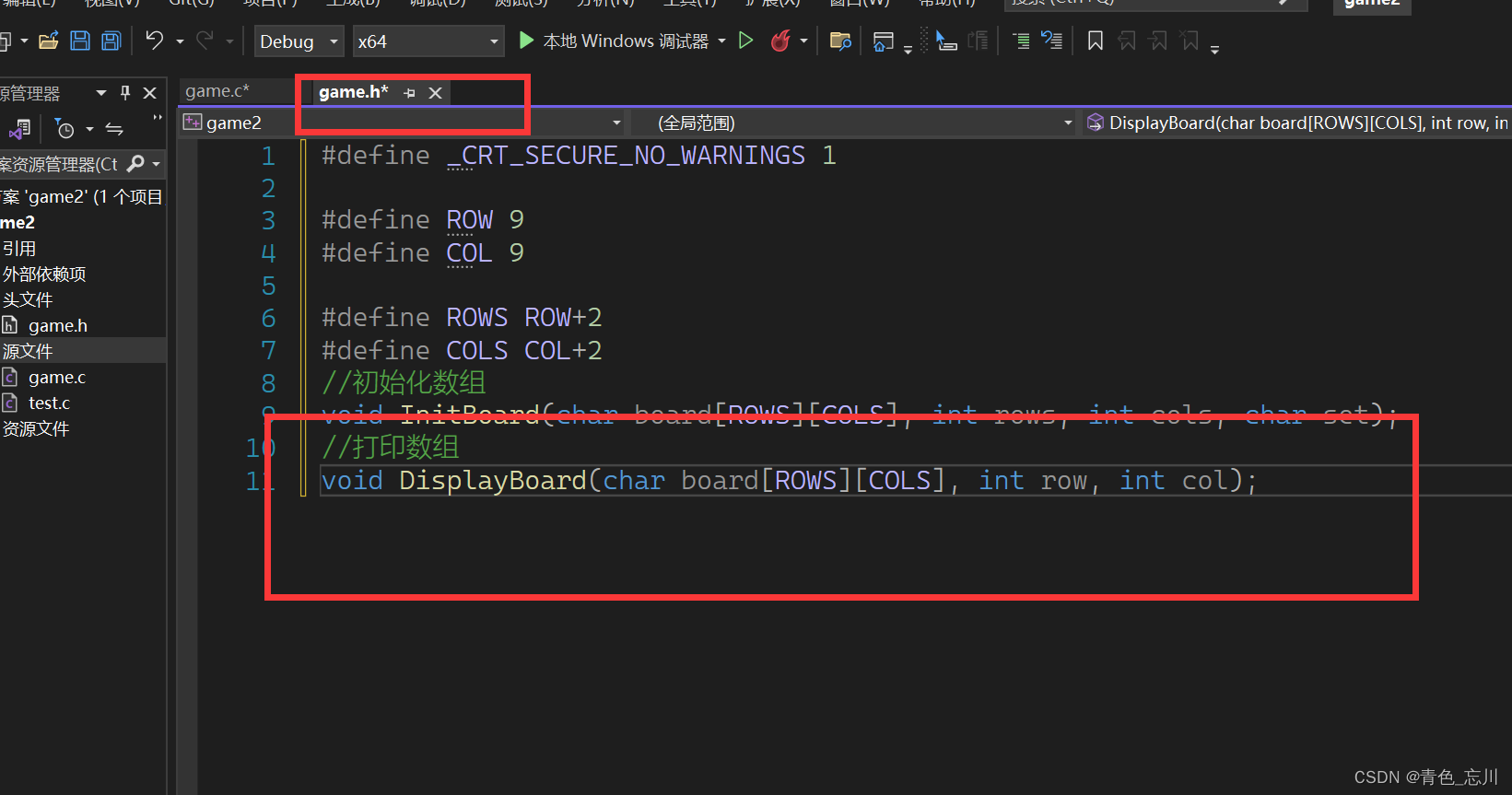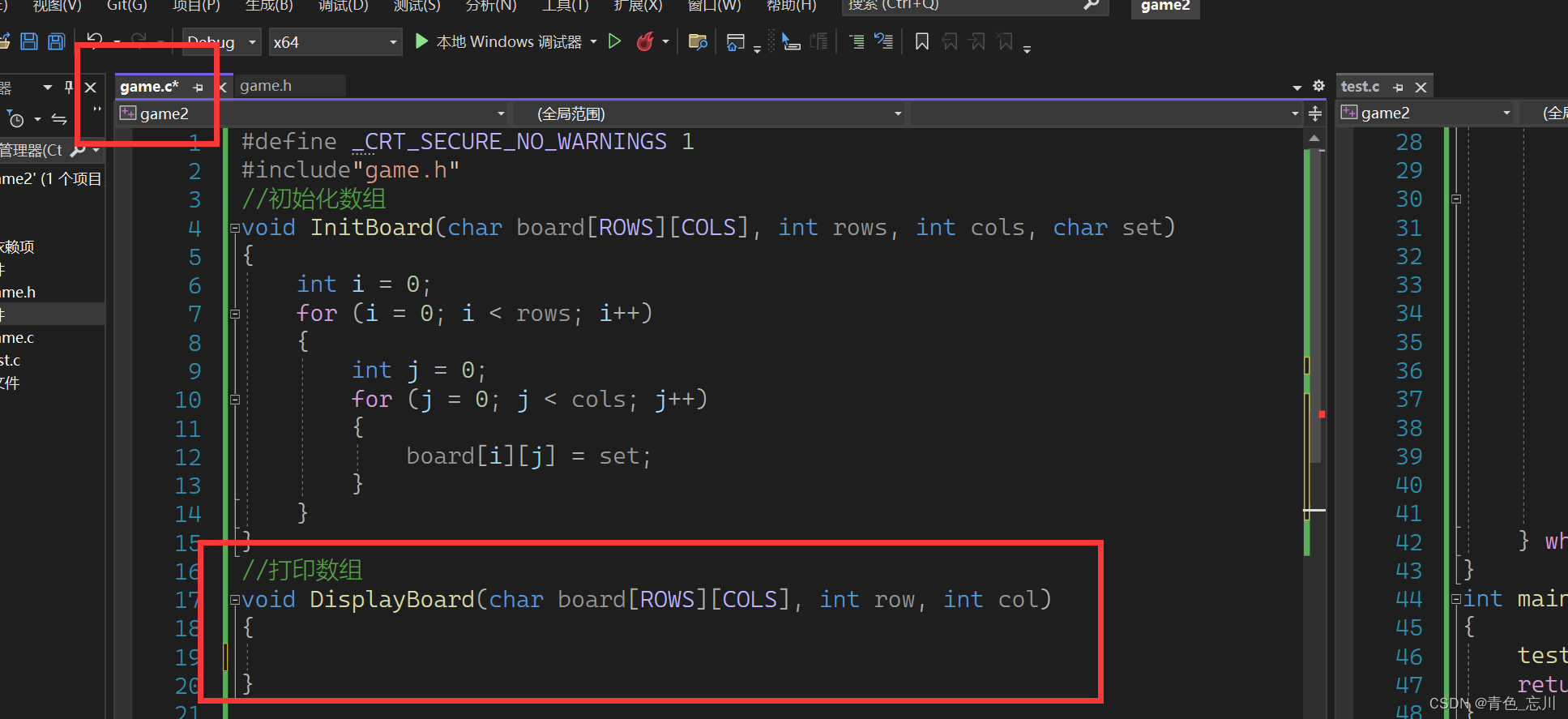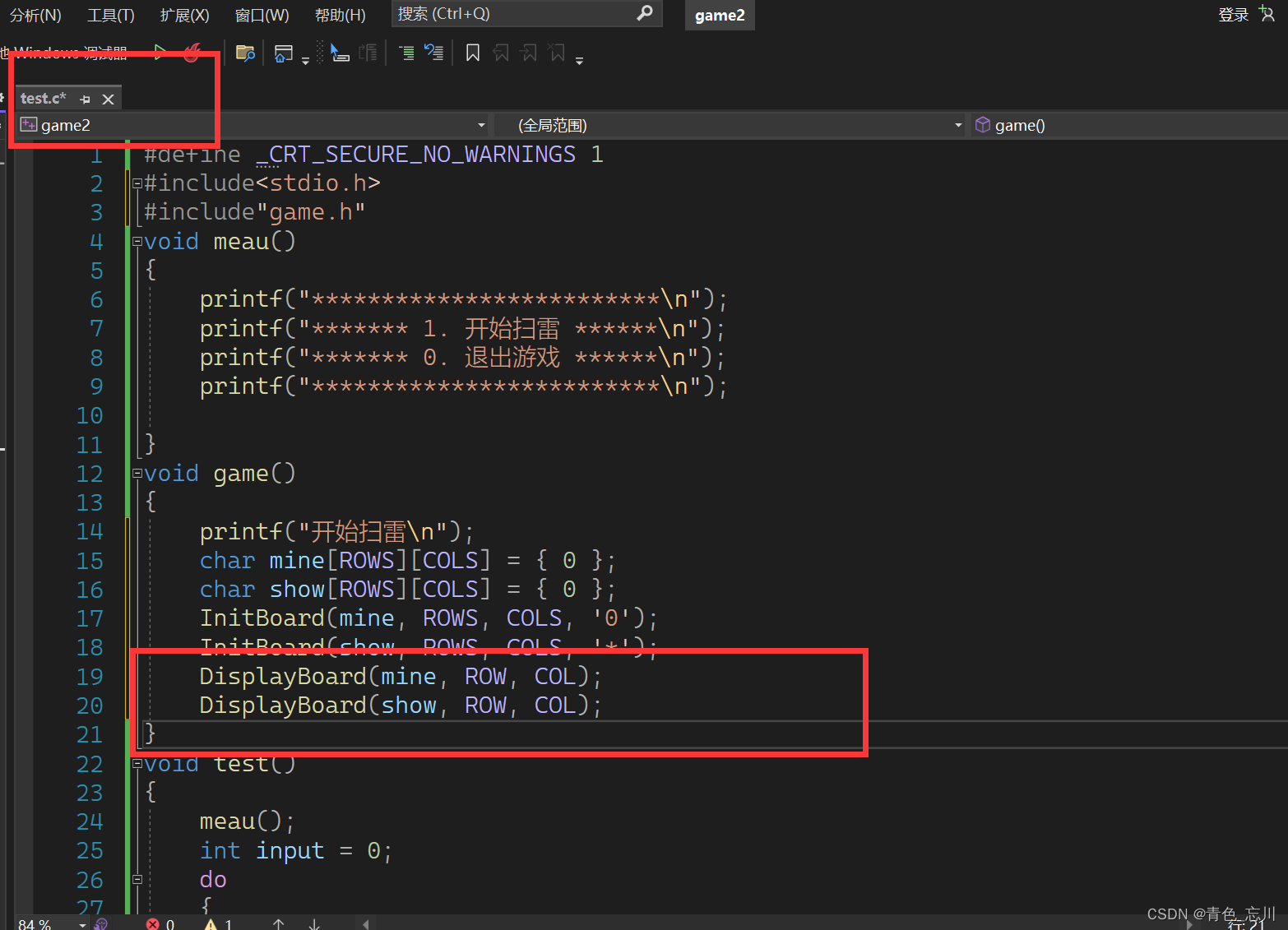### （2）代码的实现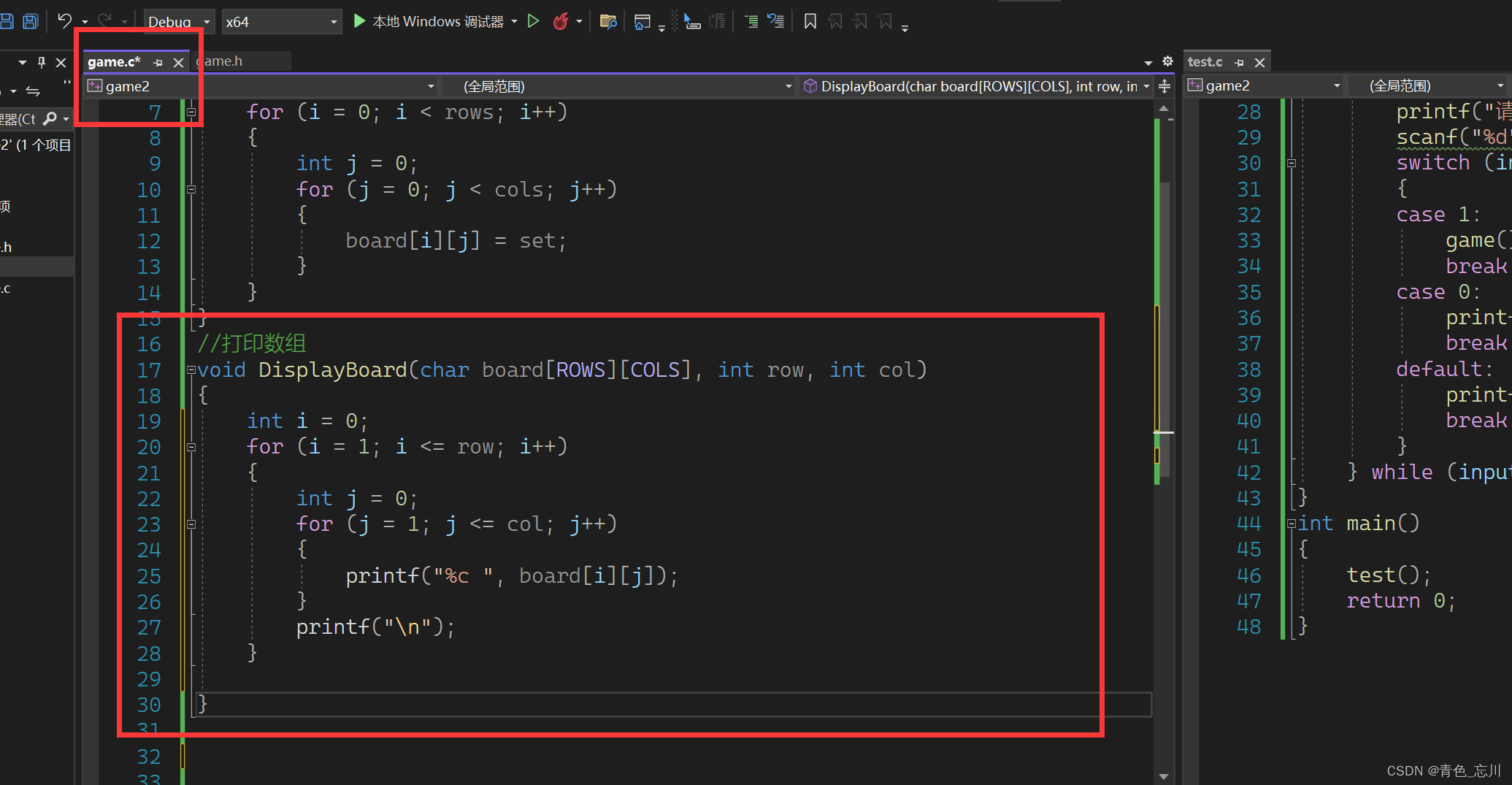### （3）测试代码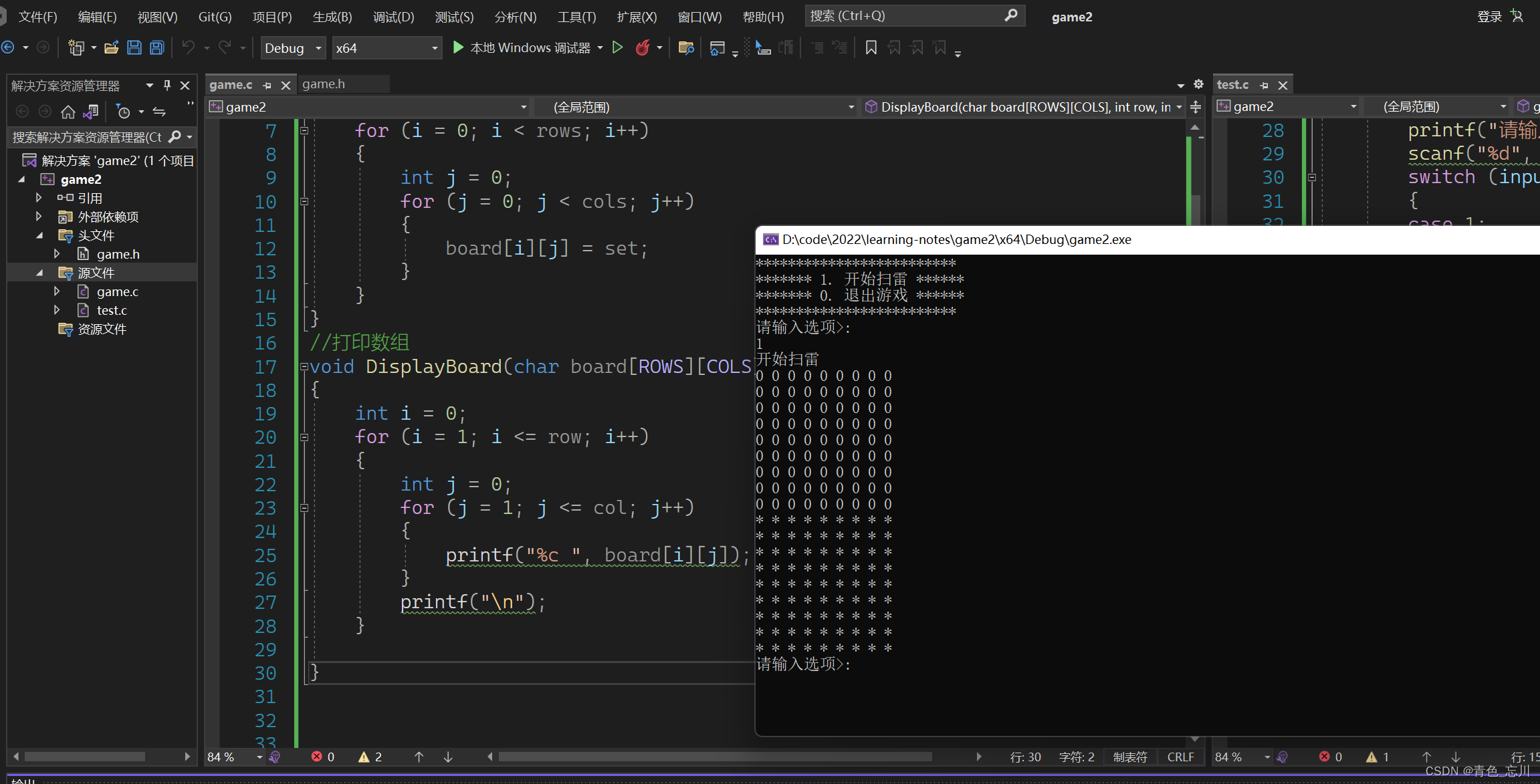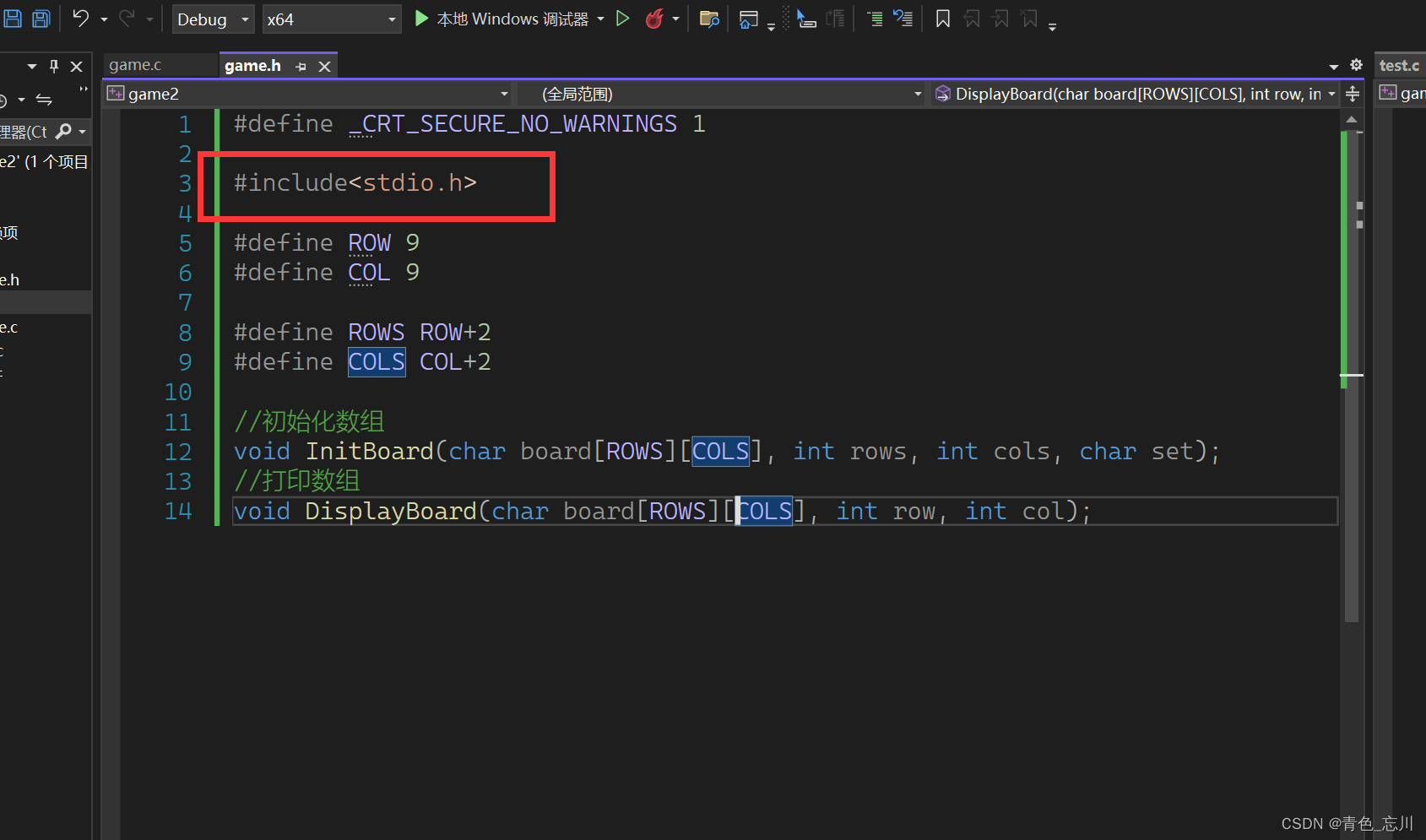### （4）更进一步优化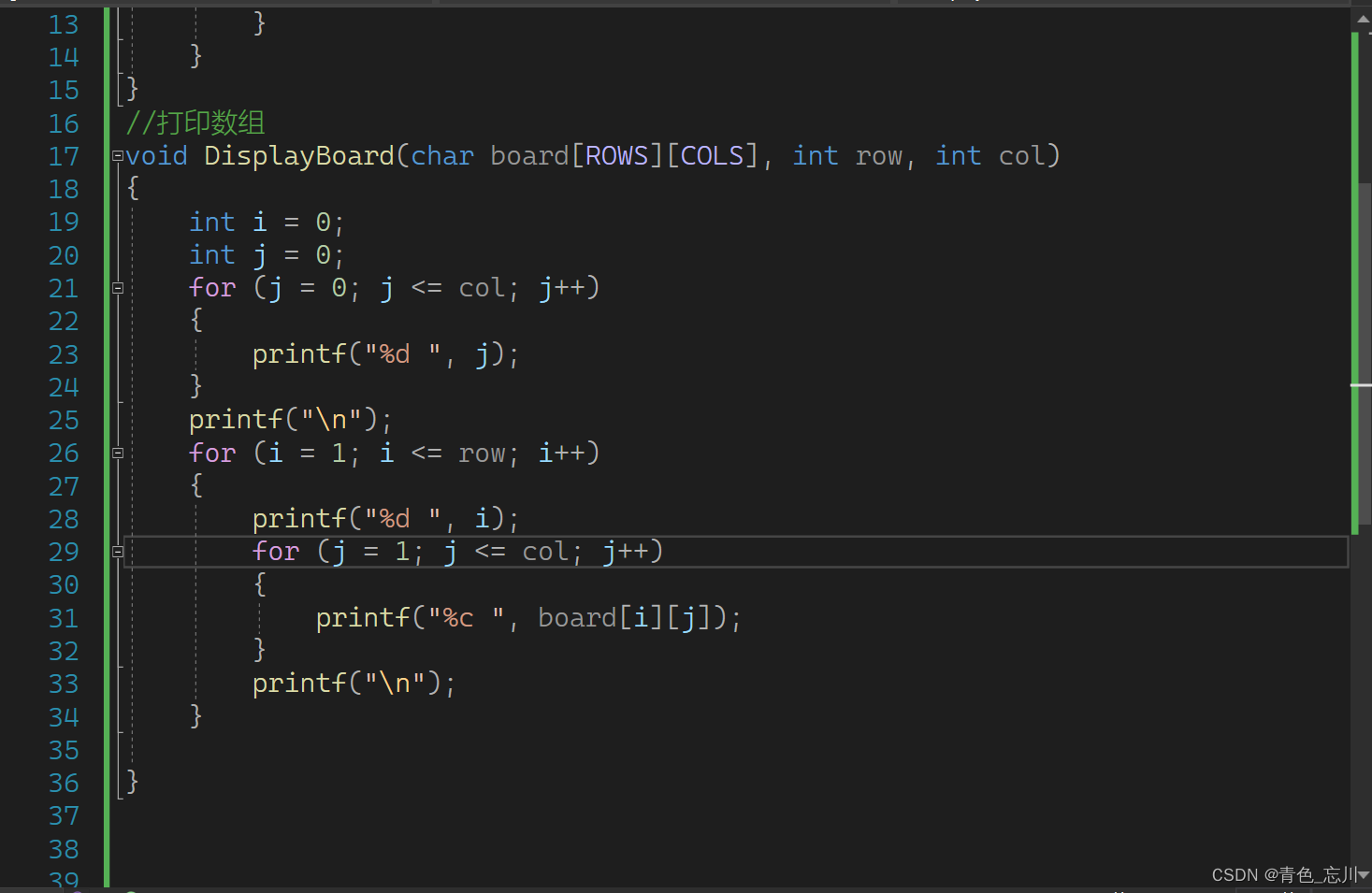### （5）运行测试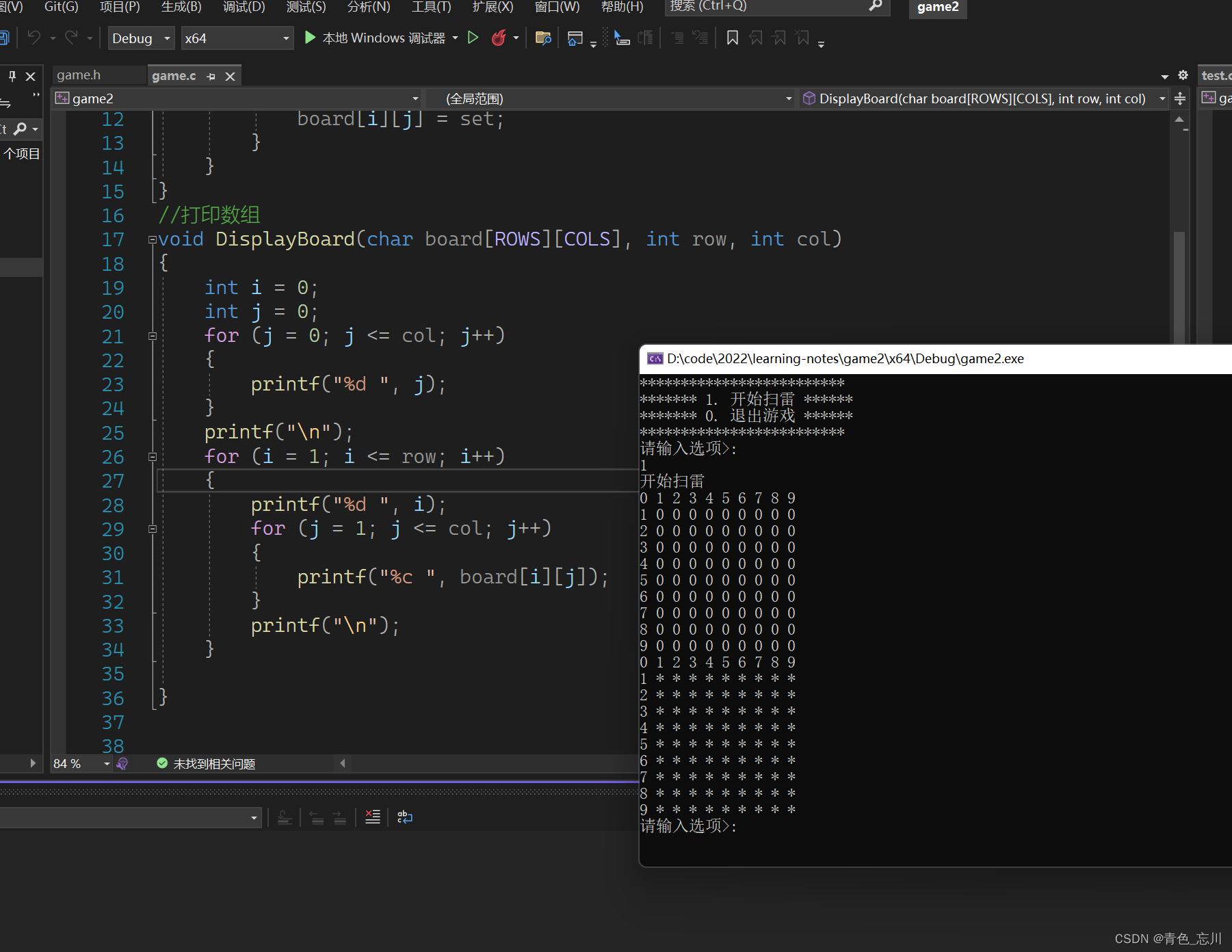### （6）更进一步的优化界面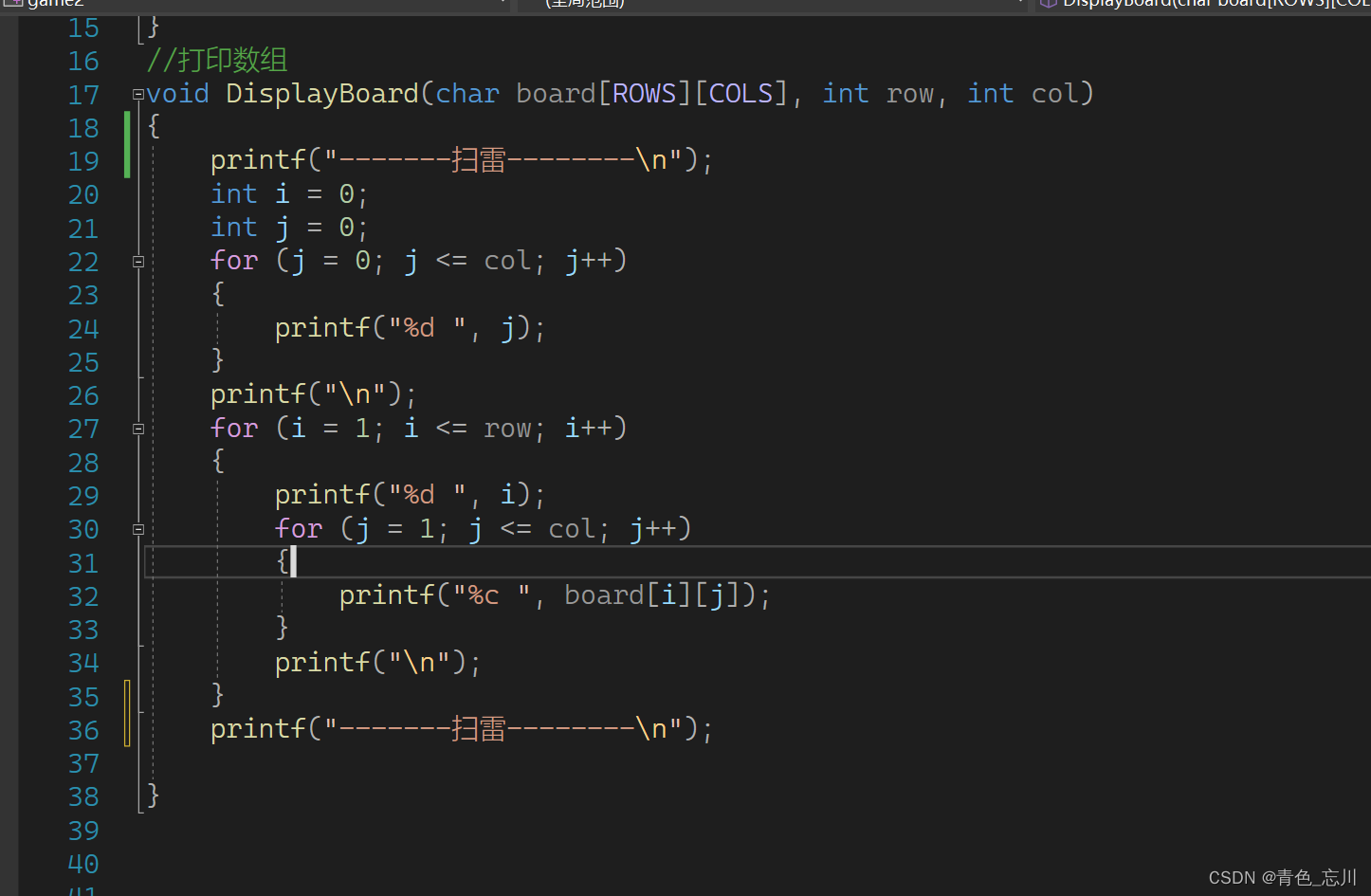这样可以使得测试更好看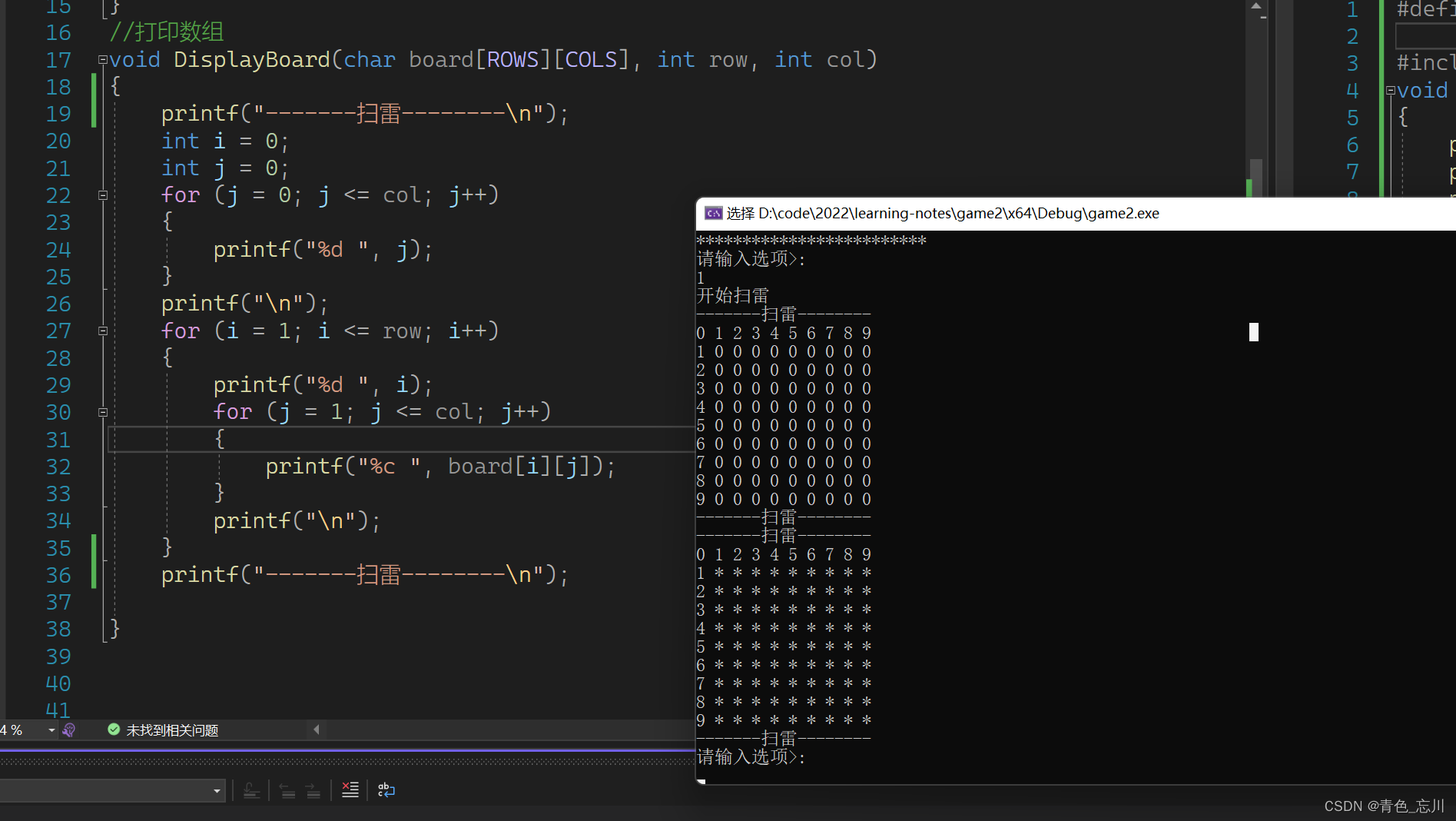### （7）代码实现如下

``````//打印数组
void DisplayBoard(char board[ROWS][COLS], int row, int col)
{
printf("-------扫雷--------\n");
int i = 0;
int j = 0;
for (j = 0; j <= col; j++)
{
printf("%d ", j);
}
printf("\n");
for (i = 1; i <= row; i++)
{
printf("%d ", i);
for (j = 1; j <= col; j++)
{
printf("%c ", board[i][j]);
}
printf("\n");
}
printf("-------扫雷--------\n");

}
``````

## 3.布置雷(SetMine)

### (1)布置雷函数的定义和声明

``````void SetMine(char board[ROWS][COLS], int row, int col);
``````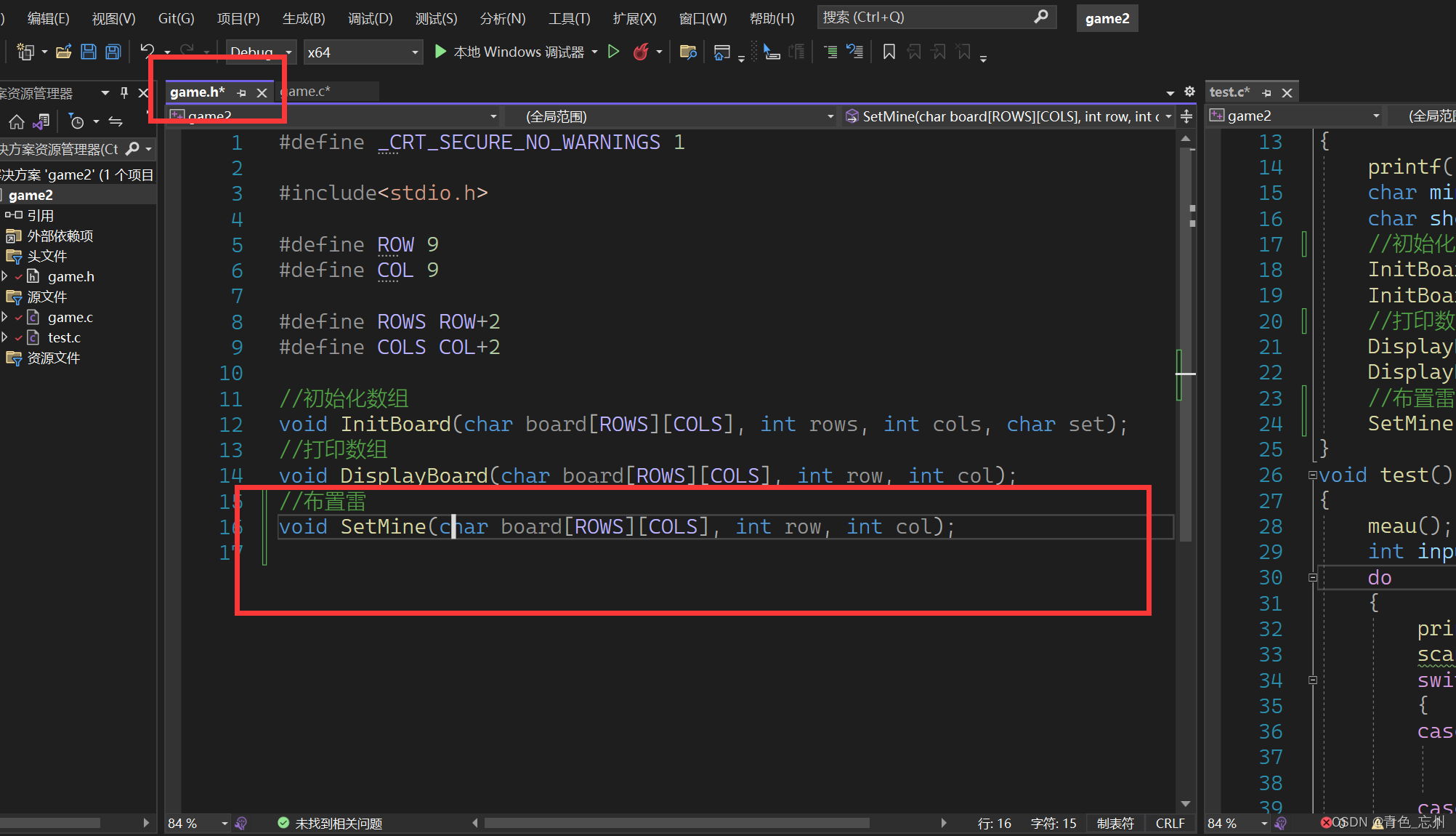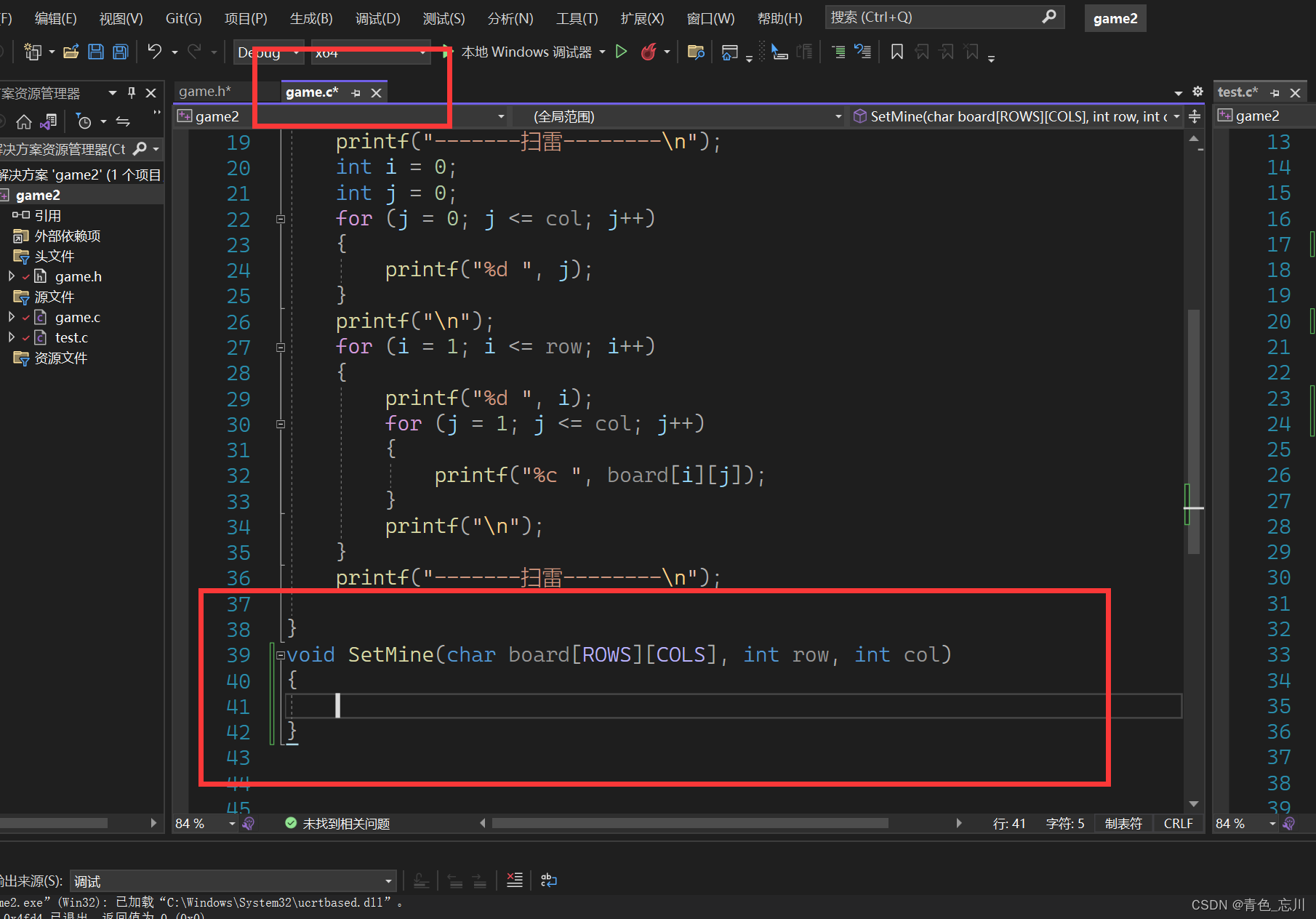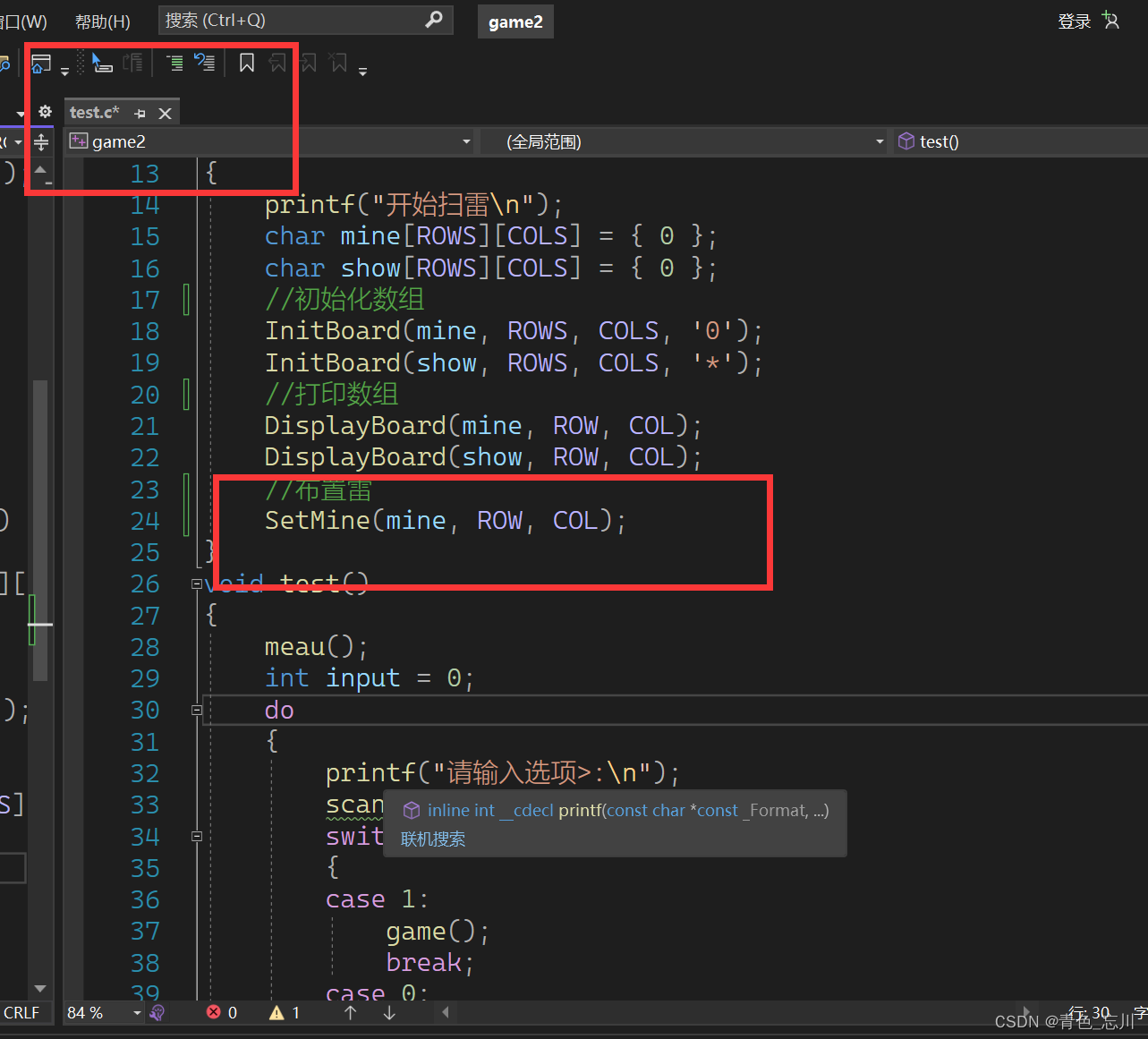（2）雷的个数以及雷坐标的生成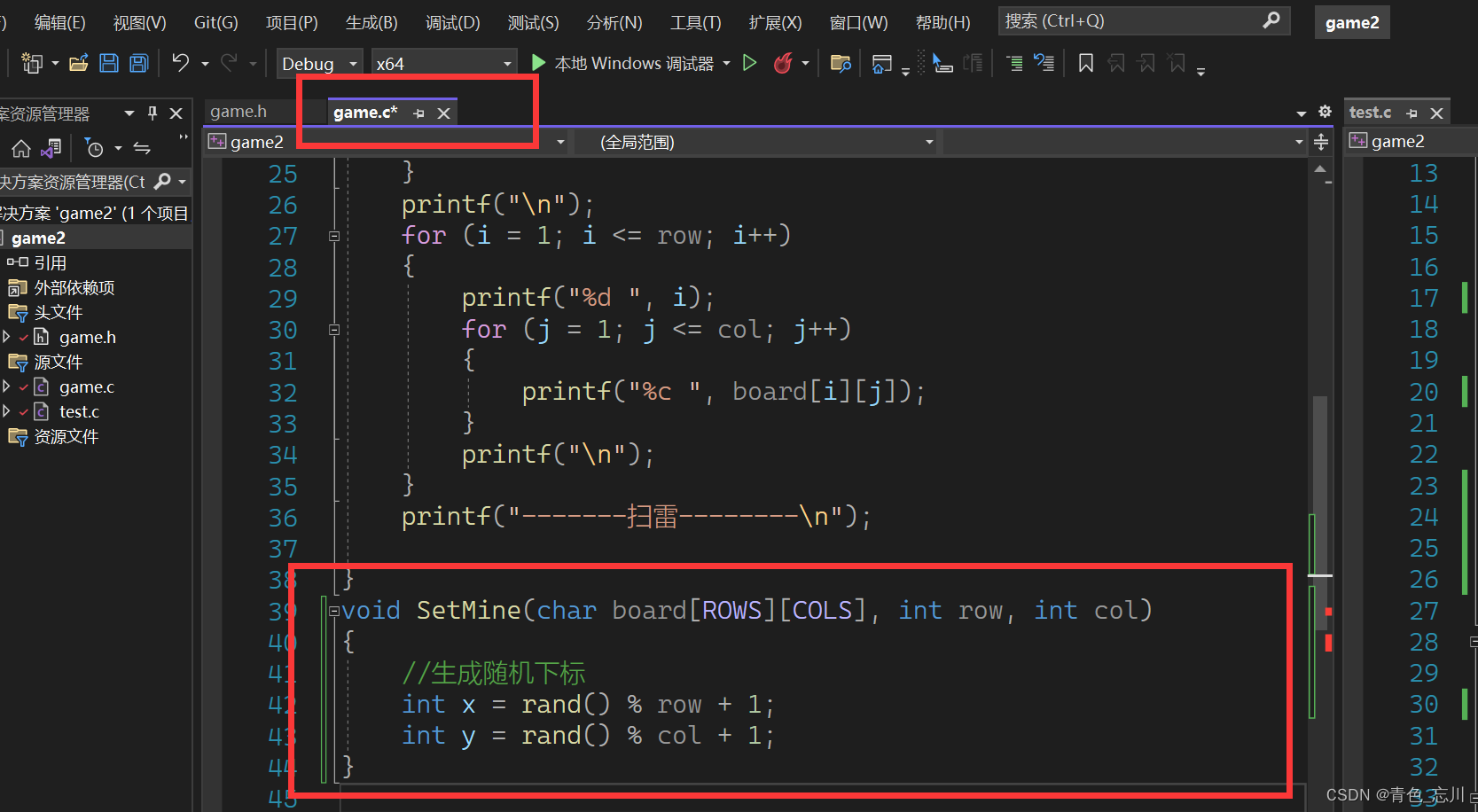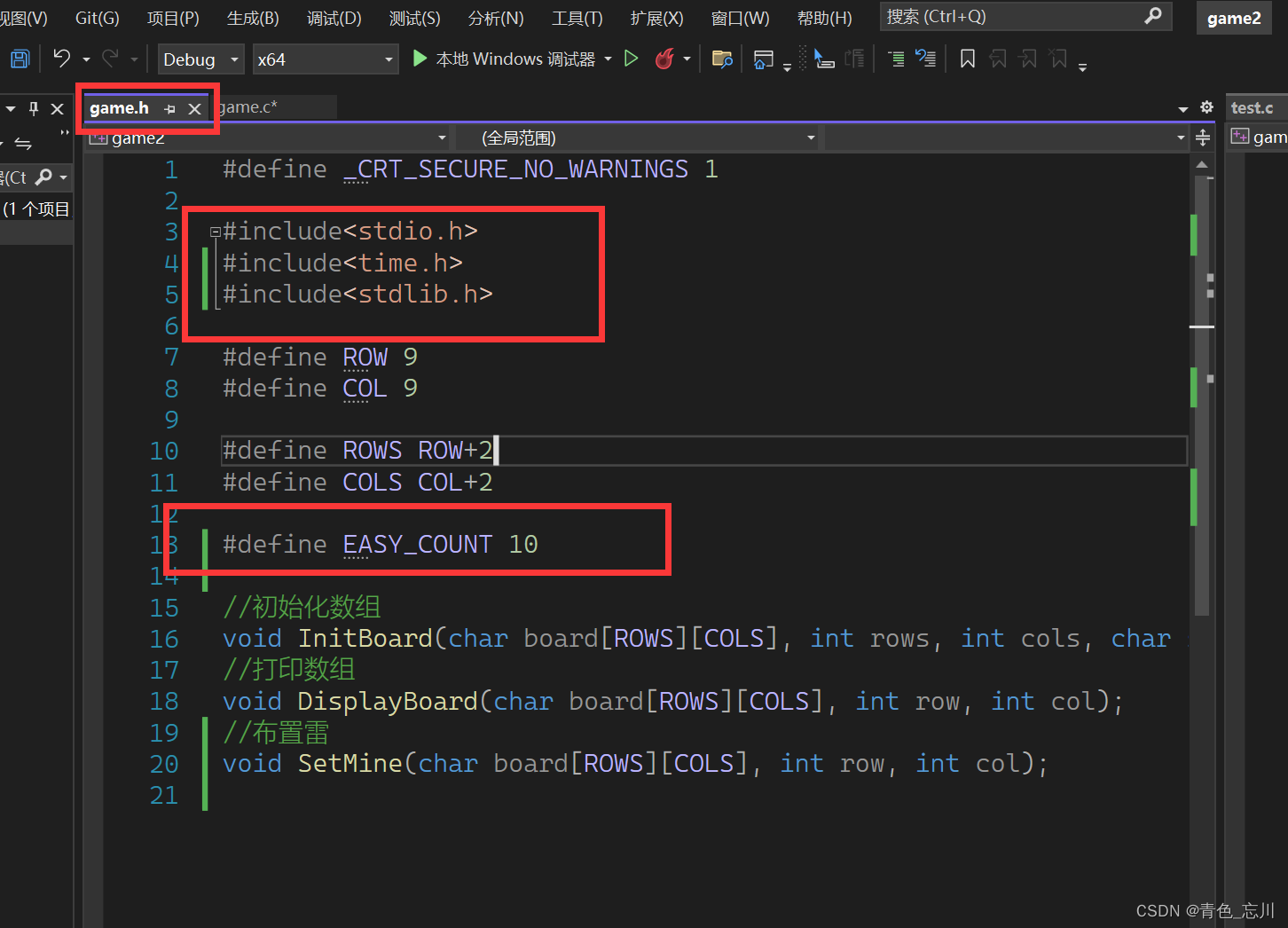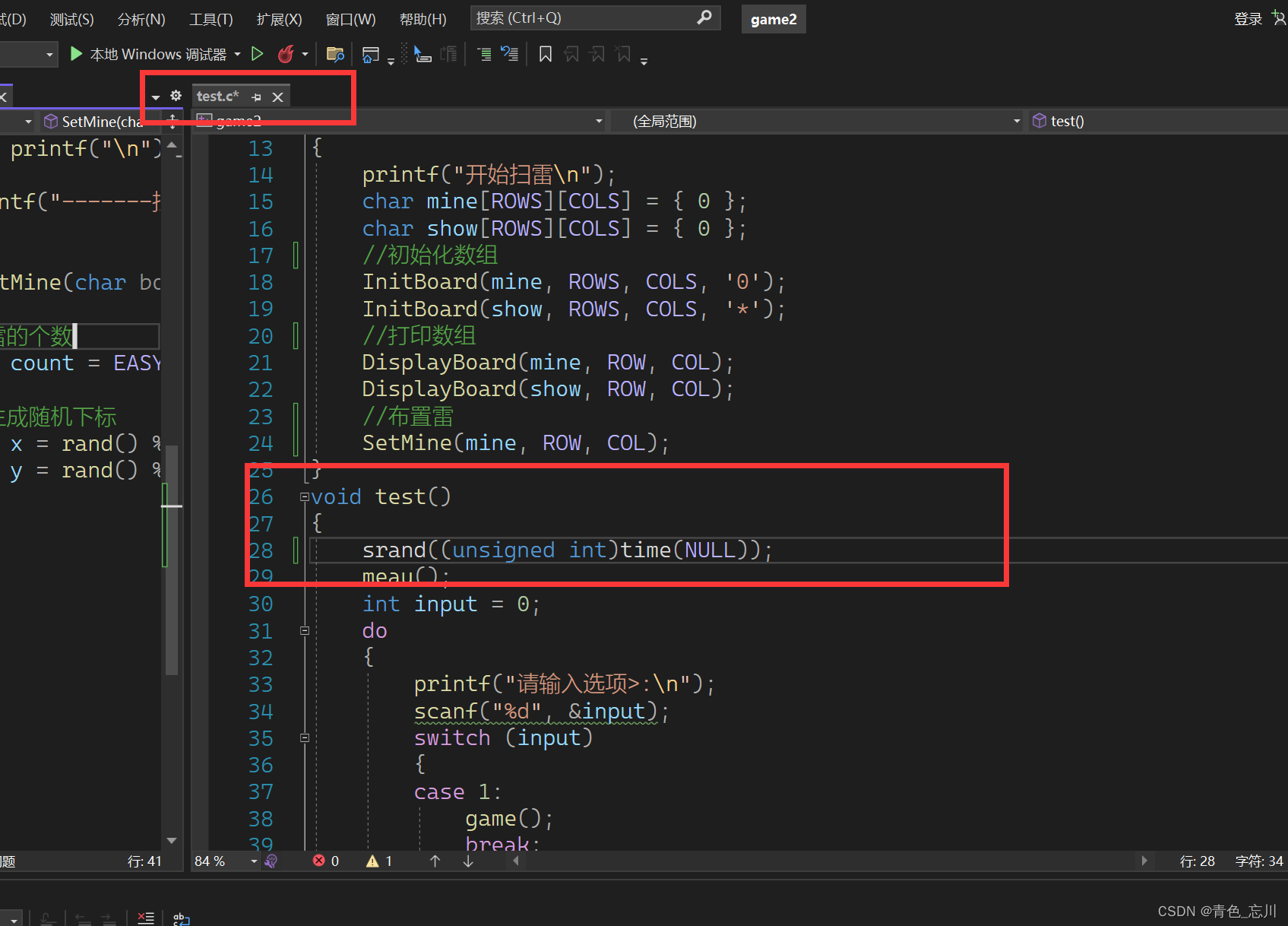### （3）具体的布置雷实现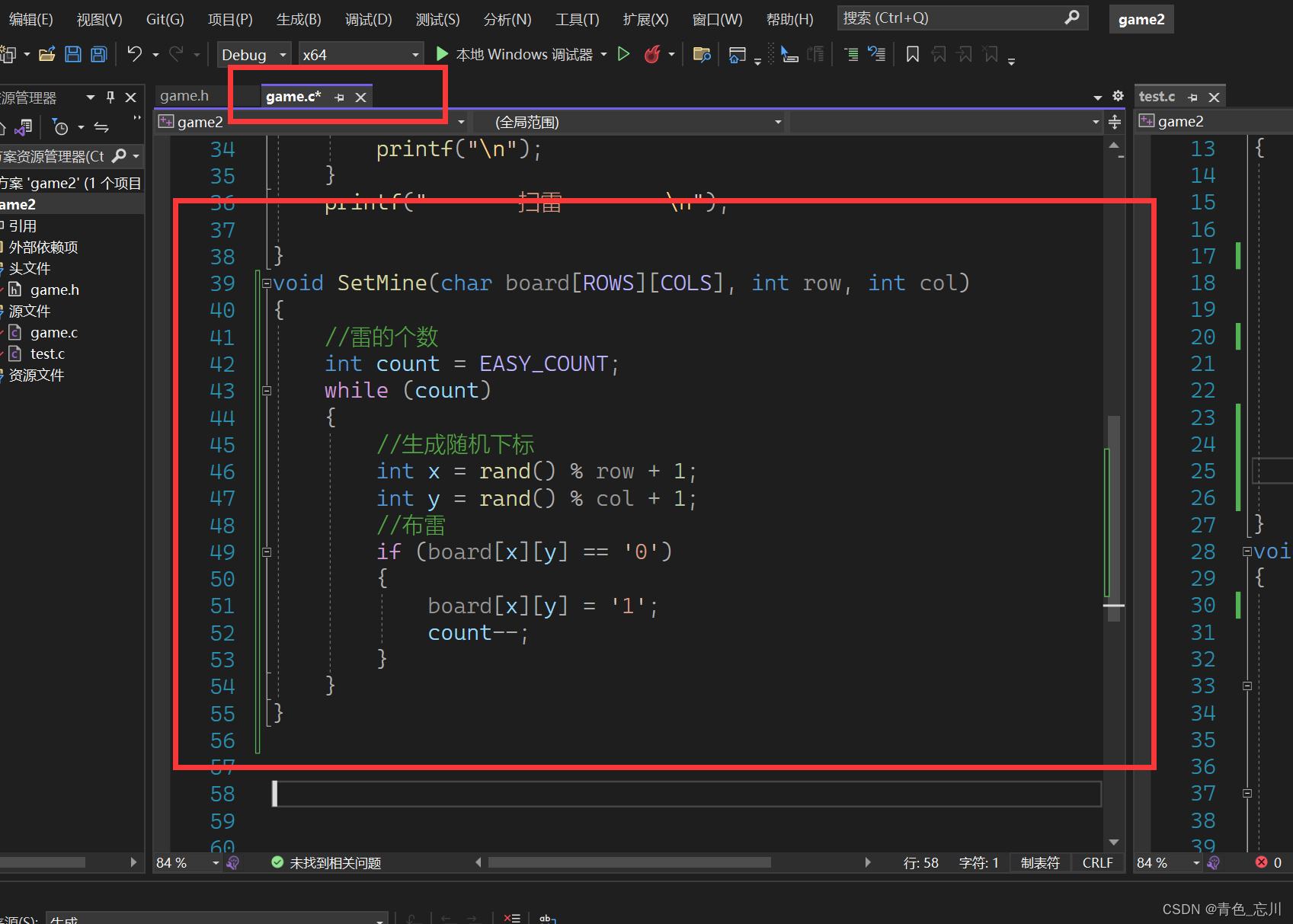### （4）测试布雷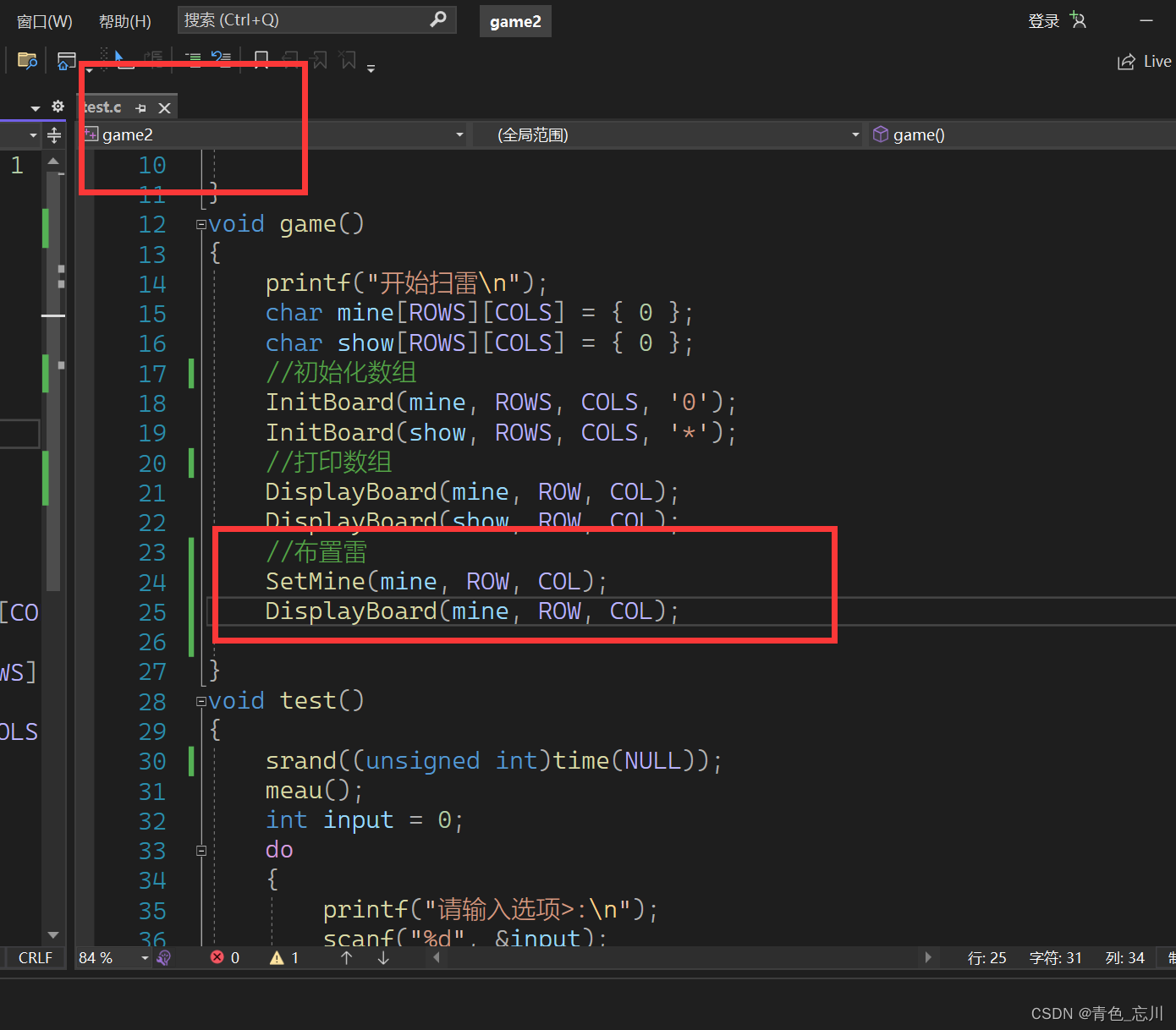结果为下图所示，刚好符合我们的预期，就是10个雷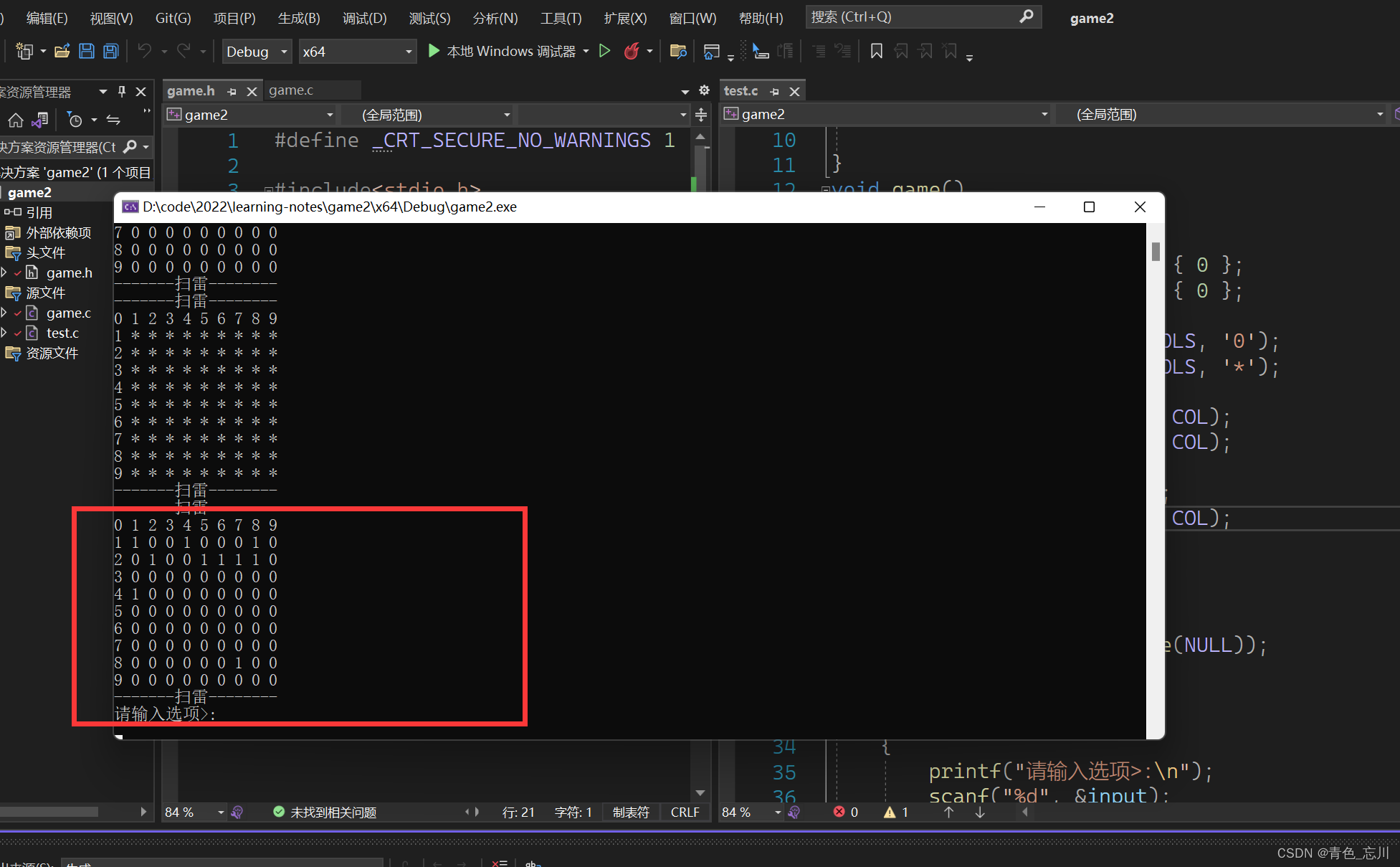### （5）布置雷的代码实现

``````//布置雷
void SetMine(char board[ROWS][COLS], int row, int col)
{
//雷的个数
int count = EASY_COUNT;
while (count)
{
//生成随机下标
int x = rand() % row + 1;
int y = rand() % col + 1;
//布雷
if (board[x][y] == '0')
{
board[x][y] = '1';
count--;
}
}
}
``````

## 4.排查雷（FindMine)

### (1)排查雷函数的声明和定义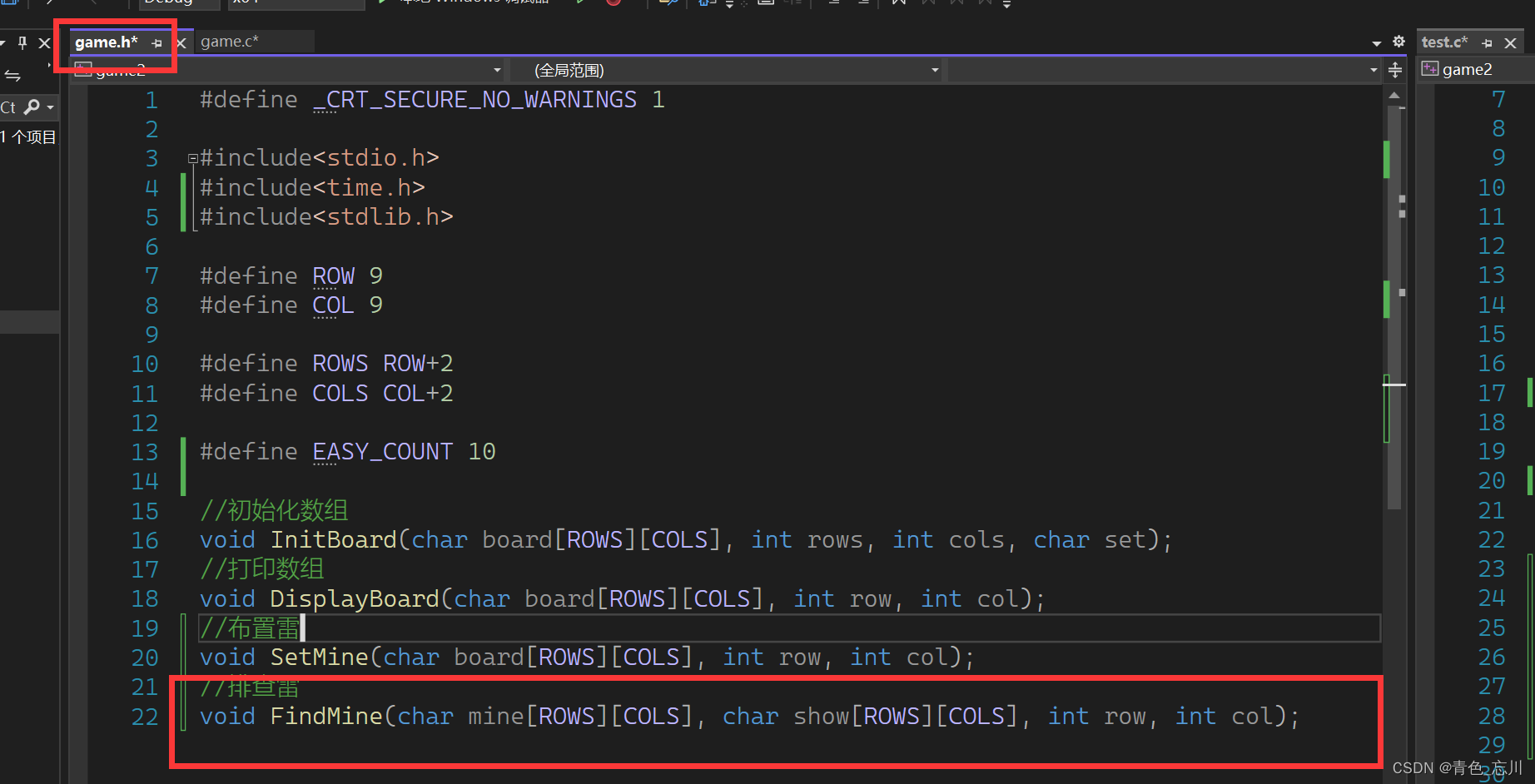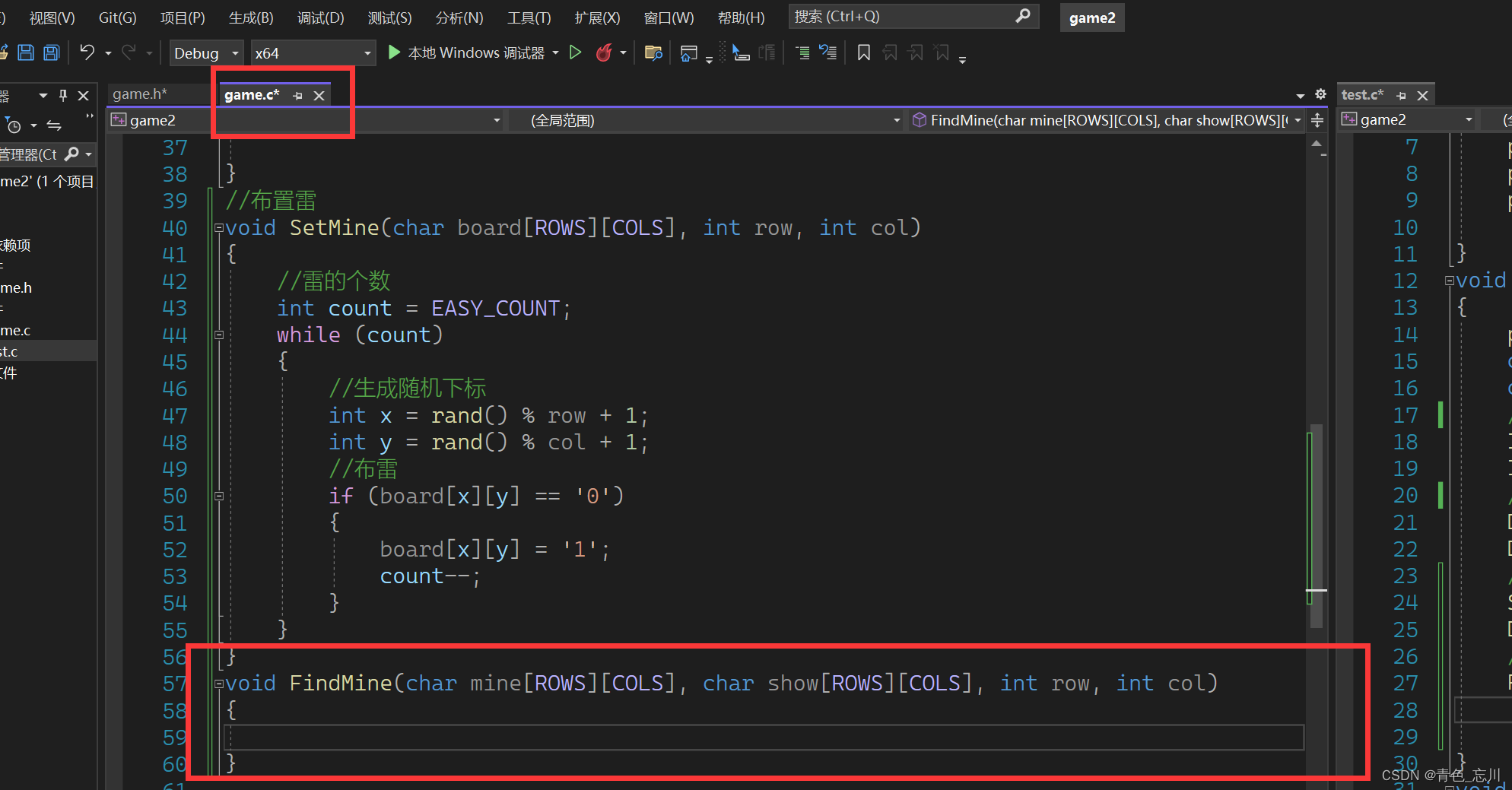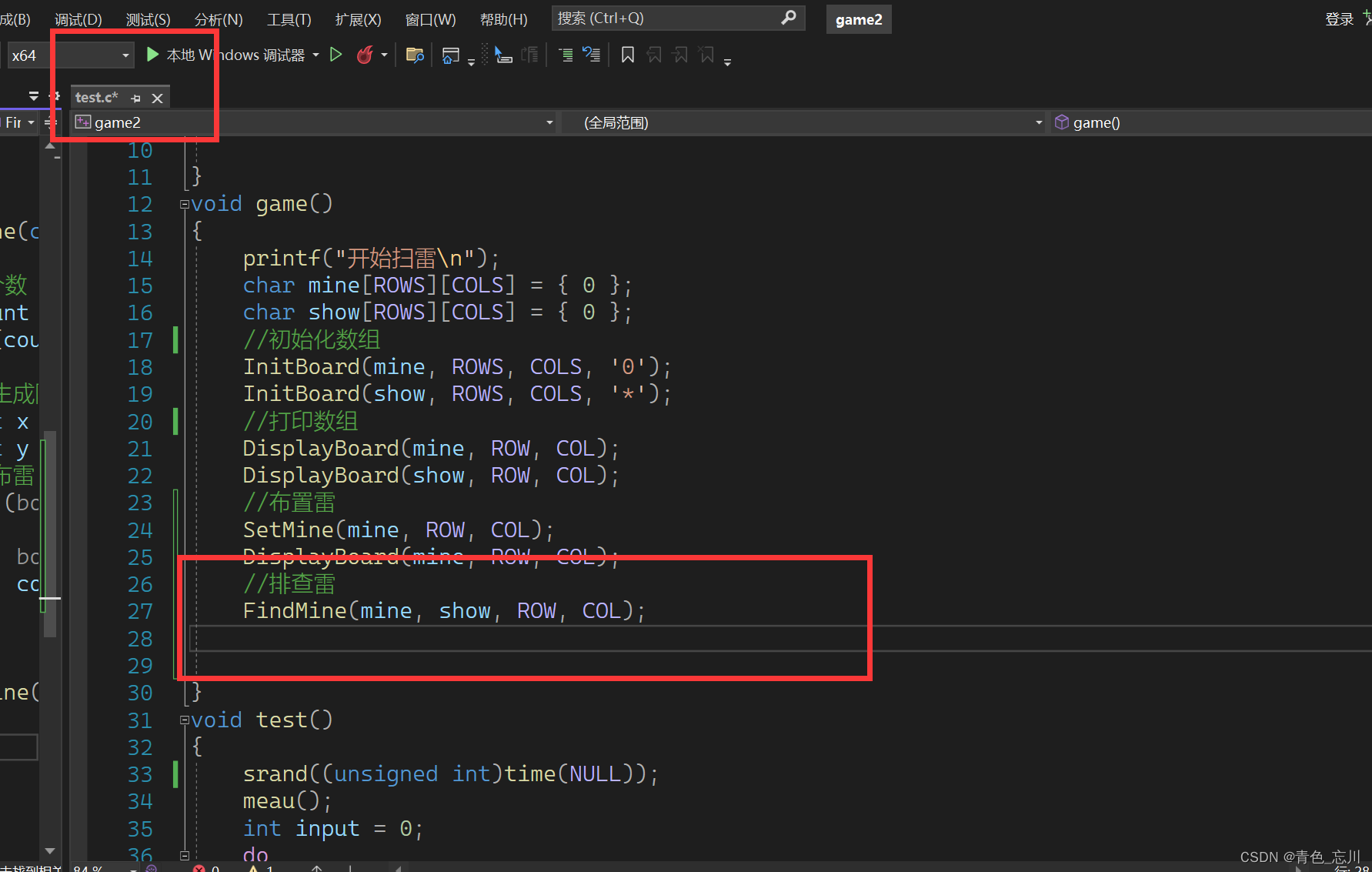### （2）排查雷的实现逻辑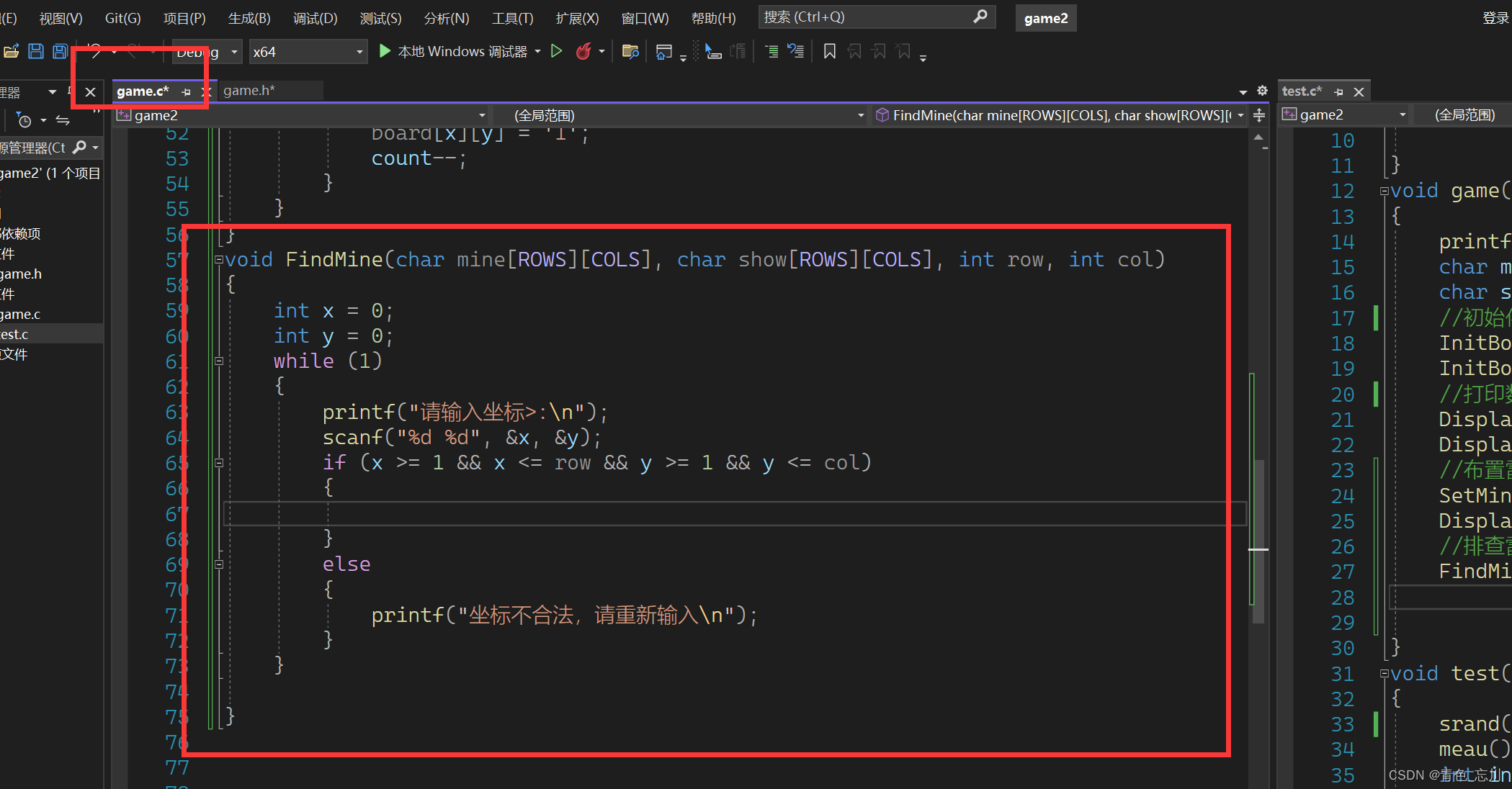现在我们可以确保我们的坐标是合法的了，在合法的基础上，如果这个坐标中的位置已经是字符1了，那玩家就被炸死了，游戏结束并打印出雷区的棋盘。如果不是字符1，那就开始统计他的一圈有多少个雷，为了方便统起见，我们使用一个函数来进行统计。这个函数我们不妨就定义为

``int get_mine_count(char mine[ROWS][COLS], int x, int y);``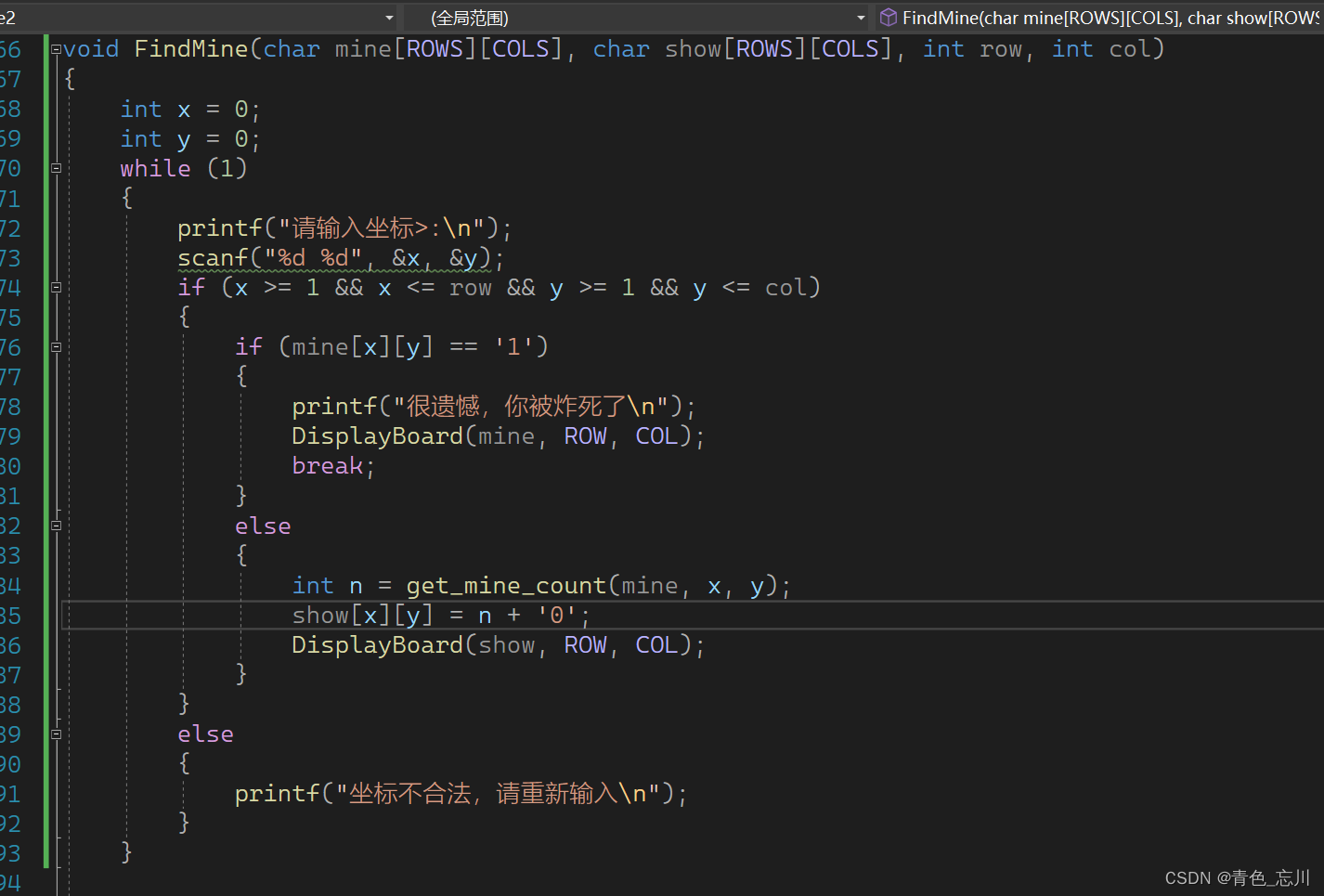那么我们现在该实现get_mine_count这个函数了，这个难度也不大，我们把周围一圈的都加起来然后减去8个字符0就可以解决了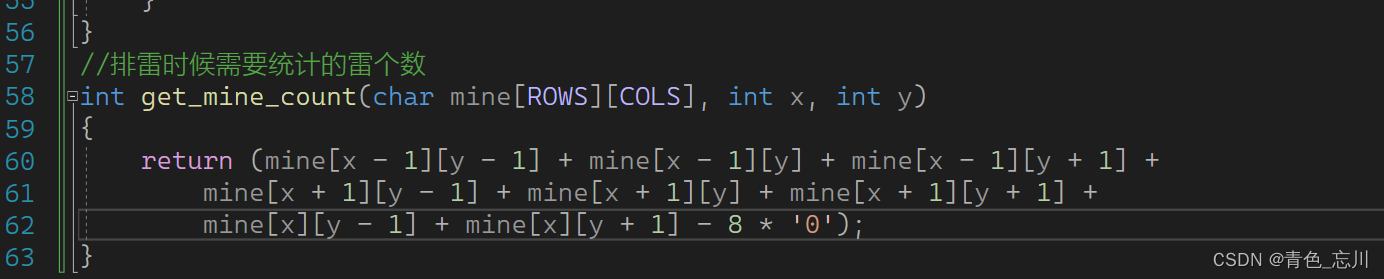### （3)测试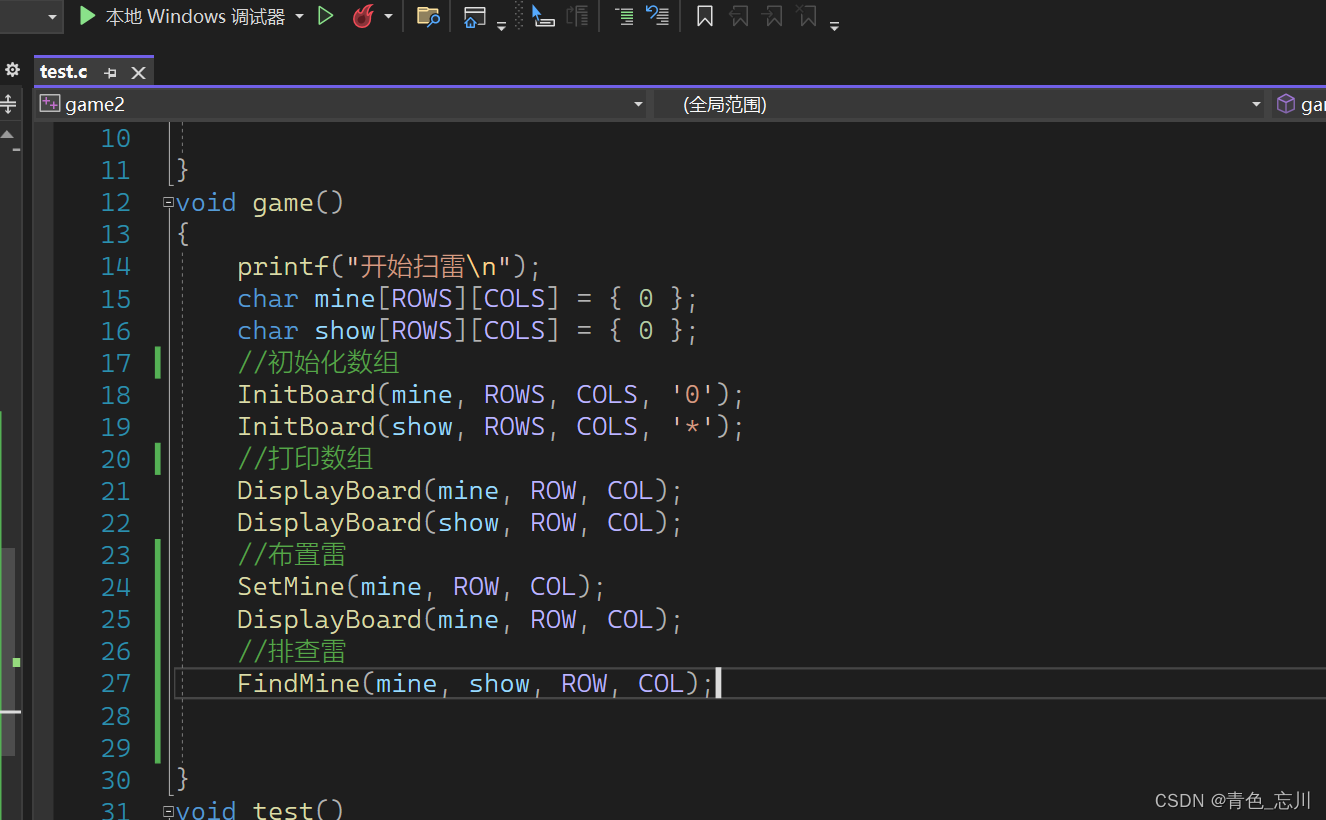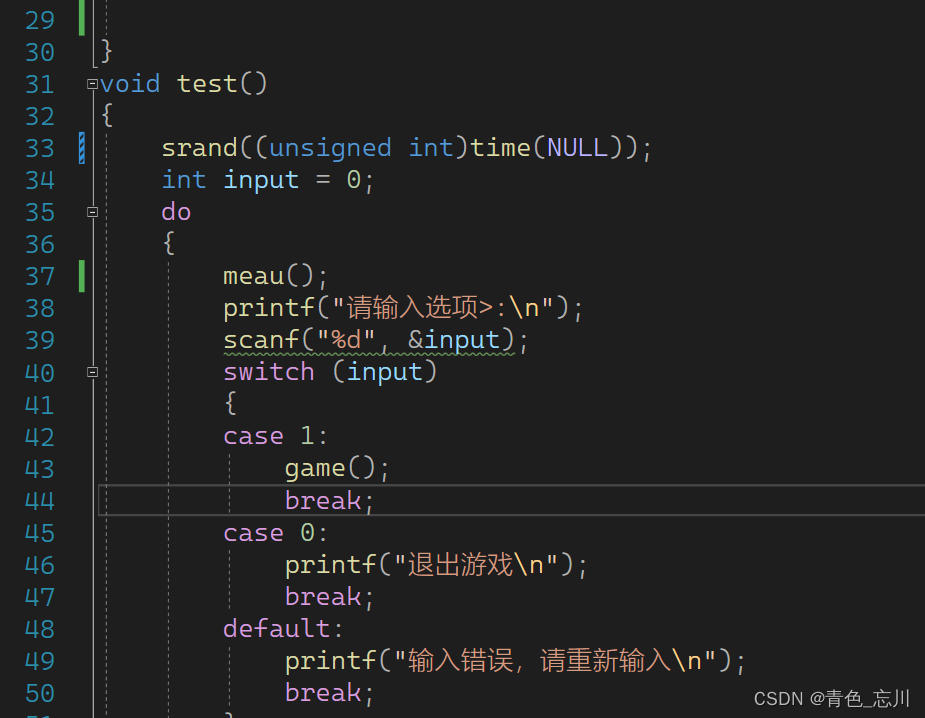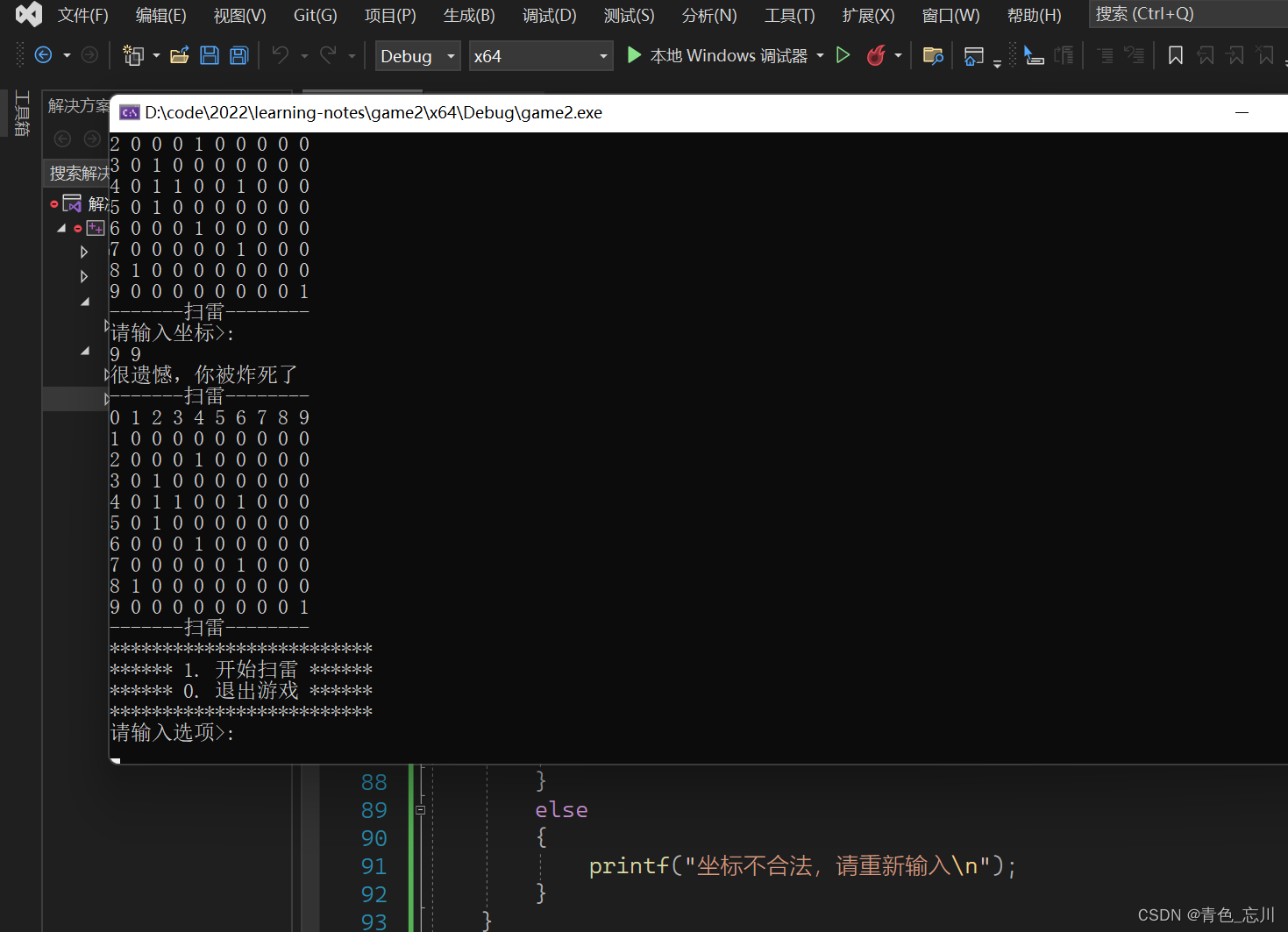坐标不合法案例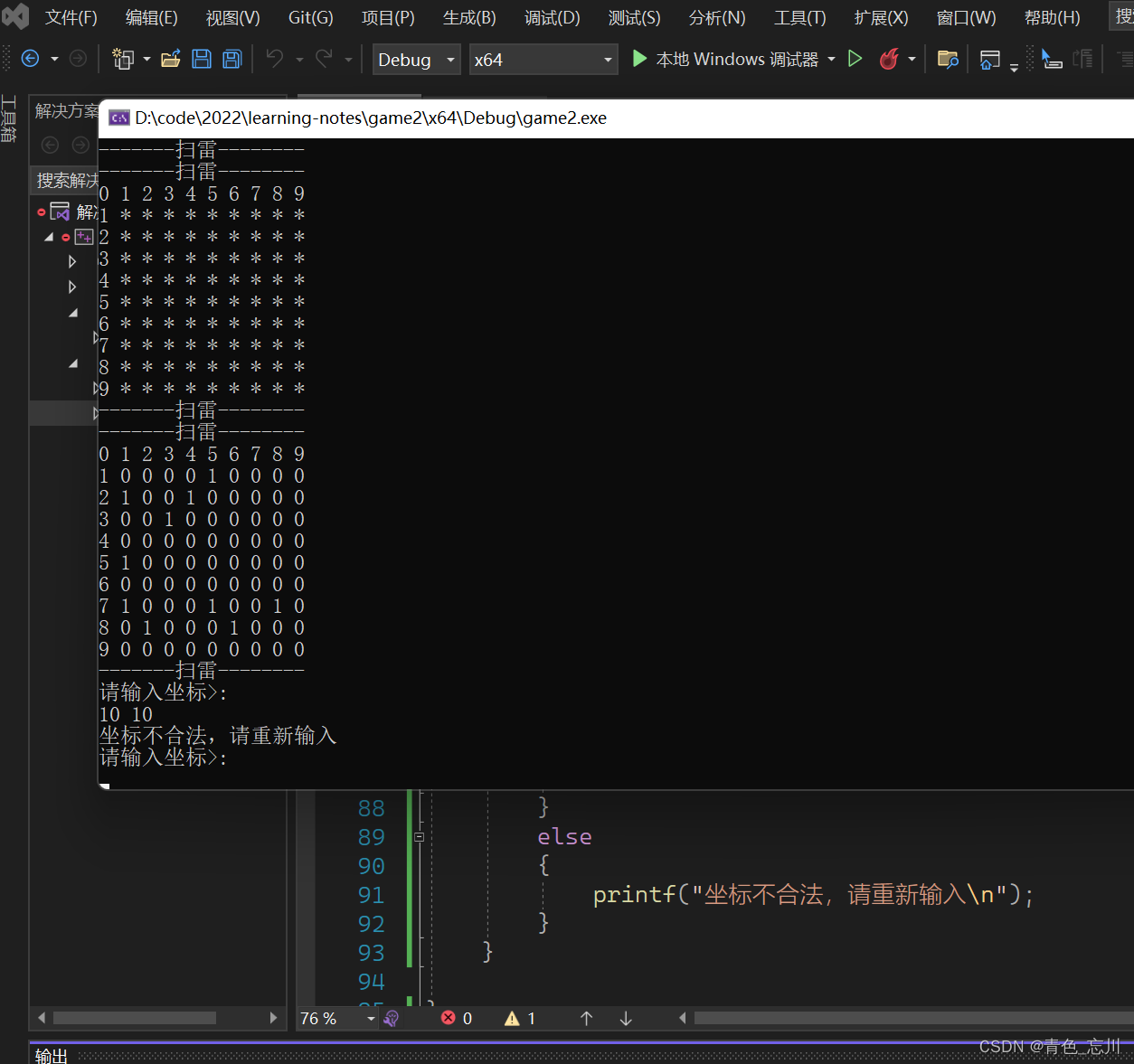### （4）排雷的结束条件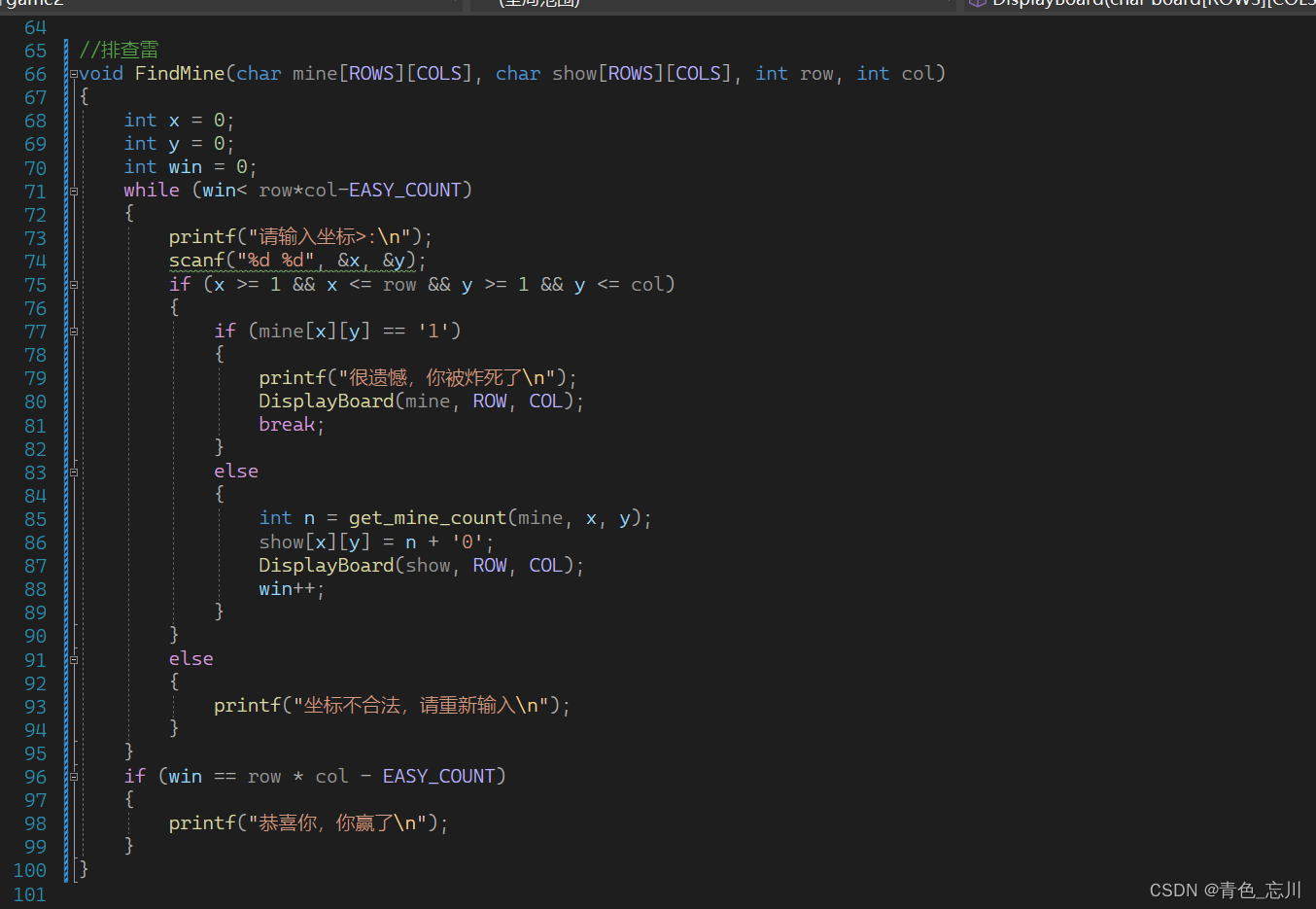为了方便测试，我们将雷的数量暂时改为80个，符合我们的预期。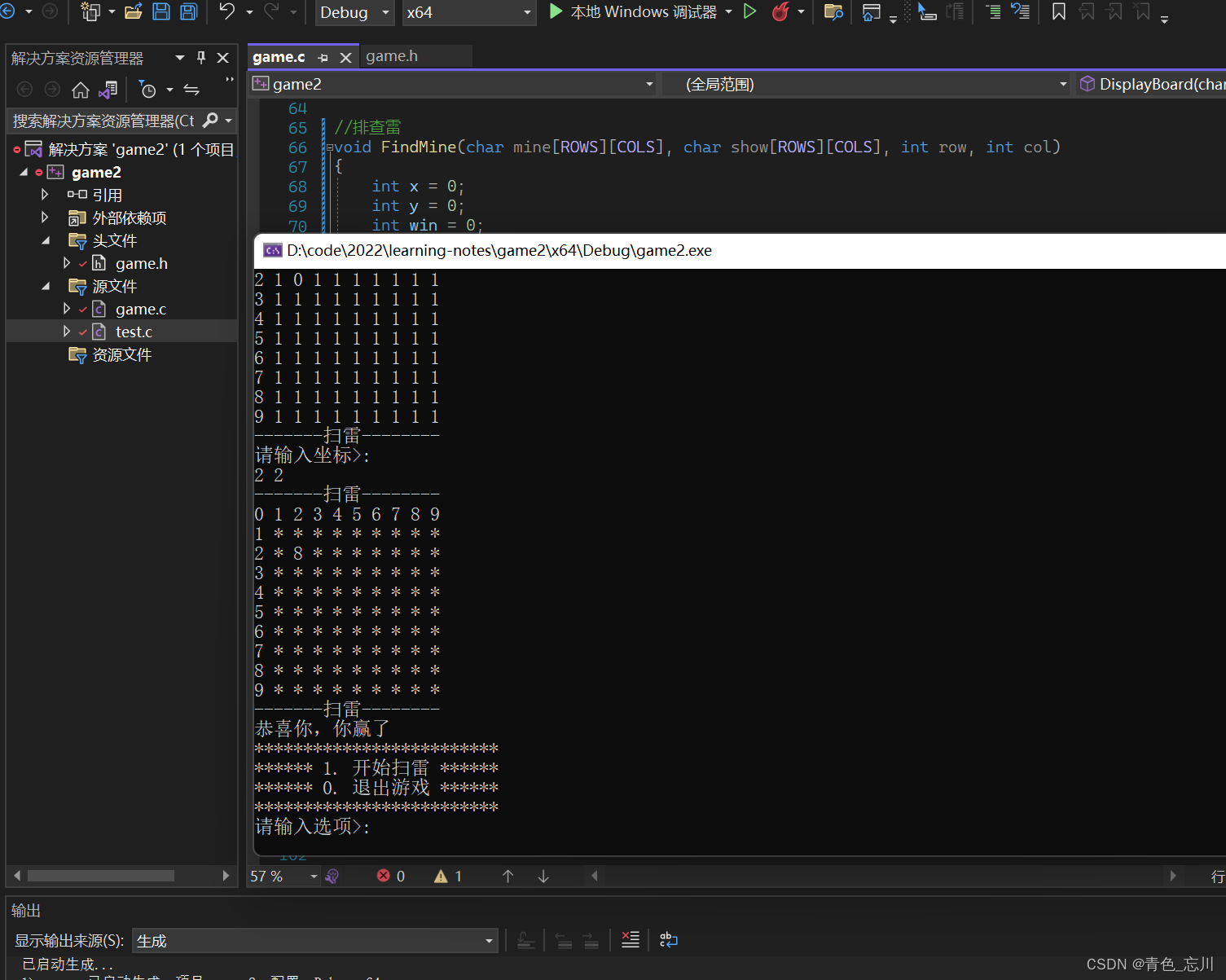### （5）优化多次排查同一个位置的bug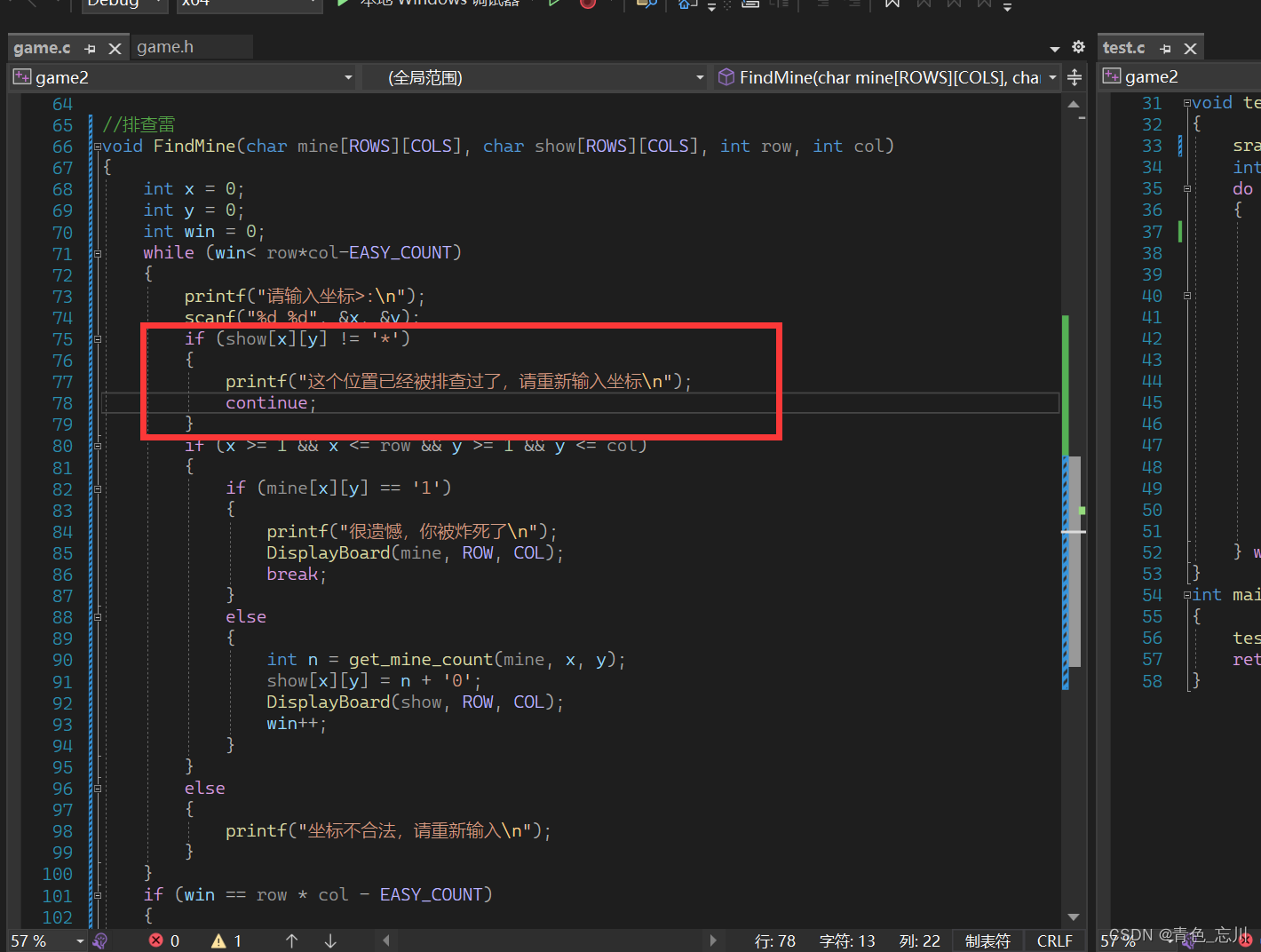下面是运行测试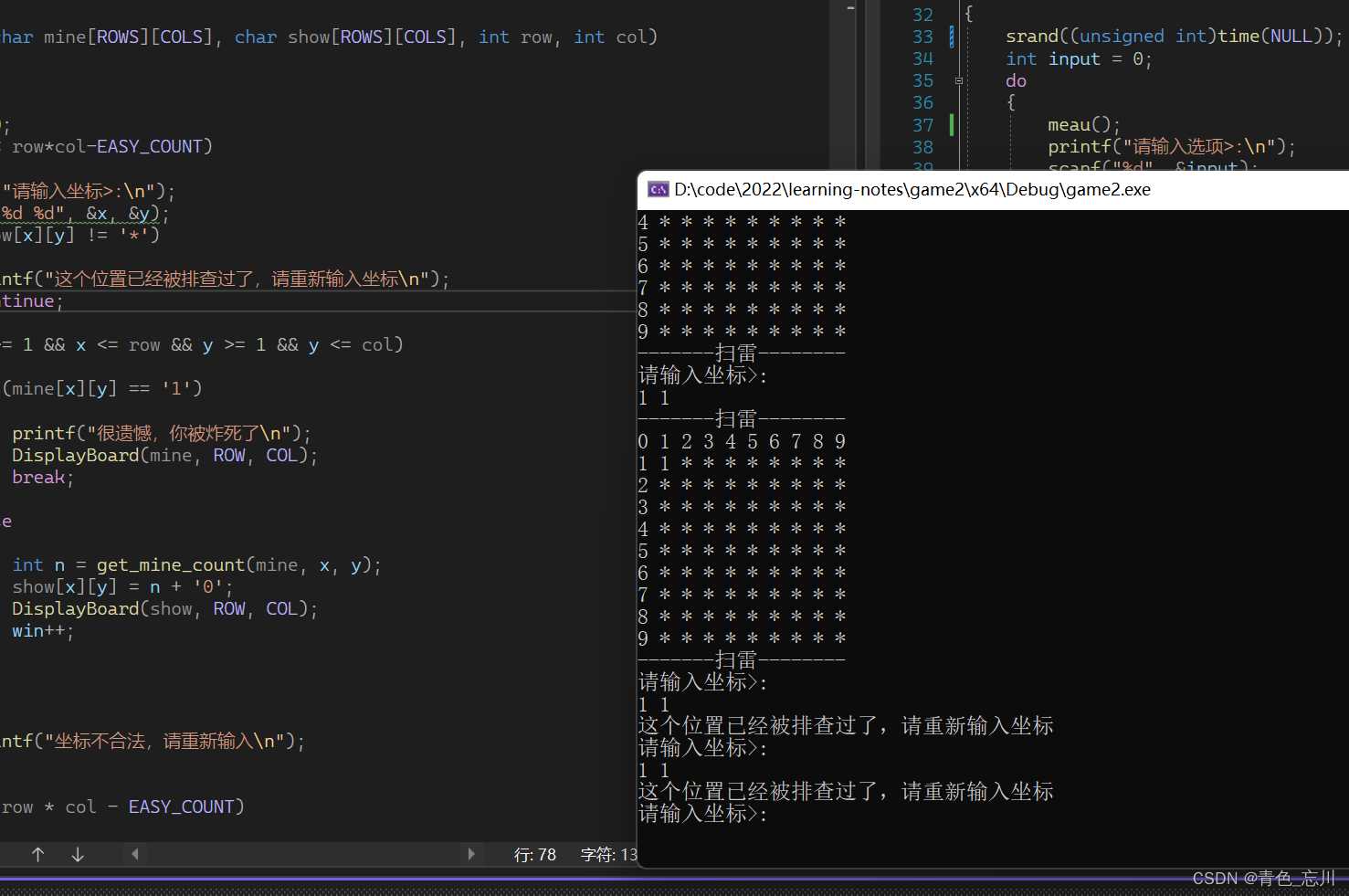### （6）排查雷代码实现如下

``````//排雷时候需要统计的雷个数
int get_mine_count(char mine[ROWS][COLS], int x, int y)
{
return (mine[x - 1][y - 1] + mine[x - 1][y] + mine[x - 1][y + 1] +
mine[x + 1][y - 1] + mine[x + 1][y] + mine[x + 1][y + 1] +
mine[x][y - 1] + mine[x][y + 1] - 8 * '0');
}

//排查雷
void FindMine(char mine[ROWS][COLS], char show[ROWS][COLS], int row, int col)
{
int x = 0;
int y = 0;
int win = 0;
while (win< row*col-EASY_COUNT)
{
printf("请输入坐标>:\n");
scanf("%d %d", &x, &y);
if (show[x][y] != '*')
{
printf("这个位置已经被排查过了，请重新输入坐标\n");
continue;
}
if (x >= 1 && x <= row && y >= 1 && y <= col)
{
if (mine[x][y] == '1')
{
printf("很遗憾，你被炸死了\n");
DisplayBoard(mine, ROW, COL);
break;
}
else
{
int n = get_mine_count(mine, x, y);
show[x][y] = n + '0';
DisplayBoard(show, ROW, COL);
win++;
}
}
else
{
printf("坐标不合法，请重新输入\n");
}
}
if (win == row * col - EASY_COUNT)
{
printf("恭喜你，你赢了\n");
DisplayBoard(mine, ROW, COL);
}
}
``````

# 四、游戏完整代码（分文件）

## test.c文件

``````#define _CRT_SECURE_NO_WARNINGS 1

#include"game.h"
void meau()
{
printf("*************************\n");
printf("****** 1. 开始扫雷 ******\n");
printf("****** 0. 退出游戏 ******\n");
printf("*************************\n");

}
void game()
{
printf("开始扫雷\n");
char mine[ROWS][COLS] = { 0 };
char show[ROWS][COLS] = { 0 };
//初始化数组
InitBoard(mine, ROWS, COLS, '0');
InitBoard(show, ROWS, COLS, '*');
//打印数组
//DisplayBoard(mine, ROW, COL);//这是用来测试时使用的
DisplayBoard(show, ROW, COL);
//布置雷
SetMine(mine, ROW, COL);
//DisplayBoard(mine, ROW, COL);//这是用来测试时使用的
//排查雷
FindMine(mine, show, ROW, COL);

}
void test()
{
srand((unsigned int)time(NULL));
int input = 0;
do
{
meau();
printf("请输入选项>:\n");
scanf("%d", &input);
switch (input)
{
case 1:
game();
break;
case 0:
printf("退出游戏\n");
break;
default:
printf("输入错误，请重新输入\n");
break;
}
} while (input);
}
int main()
{
test();
return 0;
}``````

## game.h文件

``````#define _CRT_SECURE_NO_WARNINGS 1

#include<stdio.h>
#include<time.h>
#include<stdlib.h>

#define ROW 9
#define COL 9

#define ROWS ROW+2
#define COLS COL+2

#define EASY_COUNT 10

//初始化数组
void InitBoard(char board[ROWS][COLS], int rows, int cols, char set);
//打印数组
void DisplayBoard(char board[ROWS][COLS], int row, int col);
//布置雷
void SetMine(char board[ROWS][COLS], int row, int col);
//排查雷
void FindMine(char mine[ROWS][COLS], char show[ROWS][COLS], int row, int col);``````

## game.c文件

``````#define _CRT_SECURE_NO_WARNINGS 1
#include"game.h"
//初始化数组
void InitBoard(char board[ROWS][COLS], int rows, int cols, char set)
{
int i = 0;
for (i = 0; i < rows; i++)
{
int j = 0;
for (j = 0; j < cols; j++)
{
board[i][j] = set;
}
}
}
//打印数组
void DisplayBoard(char board[ROWS][COLS], int row, int col)
{
printf("-------扫雷--------\n");
int i = 0;
int j = 0;
for (j = 0; j <= col; j++)
{
printf("%d ", j);
}
printf("\n");
for (i = 1; i <= row; i++)
{
printf("%d ", i);
for (j = 1; j <= col; j++)
{
printf("%c ", board[i][j]);
}
printf("\n");
}
printf("-------扫雷--------\n");

}
//布置雷
void SetMine(char board[ROWS][COLS], int row, int col)
{
//雷的个数
int count = EASY_COUNT;
while (count)
{
//生成随机下标
int x = rand() % row + 1;
int y = rand() % col + 1;
//布雷
if (board[x][y] == '0')
{
board[x][y] = '1';
count--;
}
}
}
//排雷时候需要统计的雷个数
int get_mine_count(char mine[ROWS][COLS], int x, int y)
{
return (mine[x - 1][y - 1] + mine[x - 1][y] + mine[x - 1][y + 1] +
mine[x + 1][y - 1] + mine[x + 1][y] + mine[x + 1][y + 1] +
mine[x][y - 1] + mine[x][y + 1] - 8 * '0');
}

//排查雷
void FindMine(char mine[ROWS][COLS], char show[ROWS][COLS], int row, int col)
{
int x = 0;
int y = 0;
int win = 0;
while (win< row*col-EASY_COUNT)
{
printf("请输入坐标>:\n");
scanf("%d %d", &x, &y);
if (show[x][y] != '*')
{
printf("这个位置已经被排查过了，请重新输入坐标\n");
continue;
}
if (x >= 1 && x <= row && y >= 1 && y <= col)
{
if (mine[x][y] == '1')
{
printf("很遗憾，你被炸死了\n");
DisplayBoard(mine, ROW, COL);
break;
}
else
{
int n = get_mine_count(mine, x, y);
show[x][y] = n + '0';
DisplayBoard(show, ROW, COL);
win++;
}
}
else
{
printf("坐标不合法，请重新输入\n");
}
}
if (win == row * col - EASY_COUNT)
{
printf("恭喜你，你赢了\n");
DisplayBoard(mine, ROW, COL);
}
}

``````

# 五、完整代码展示（合并为同一个文件）

``````#define _CRT_SECURE_NO_WARNINGS 1

#include<stdio.h>
#include<time.h>
#include<stdlib.h>

#define ROW 9
#define COL 9

#define ROWS ROW+2
#define COLS COL+2

#define EASY_COUNT 10

//初始化数组
void InitBoard(char board[ROWS][COLS], int rows, int cols, char set);
//打印数组
void DisplayBoard(char board[ROWS][COLS], int row, int col);
//布置雷
void SetMine(char board[ROWS][COLS], int row, int col);
//排查雷
void FindMine(char mine[ROWS][COLS], char show[ROWS][COLS], int row, int col);
void meau()
{
printf("*************************\n");
printf("****** 1. 开始扫雷 ******\n");
printf("****** 0. 退出游戏 ******\n");
printf("*************************\n");

}
void game()
{
printf("开始扫雷\n");
char mine[ROWS][COLS] = { 0 };
char show[ROWS][COLS] = { 0 };
//初始化数组
InitBoard(mine, ROWS, COLS, '0');
InitBoard(show, ROWS, COLS, '*');
//打印数组
//DisplayBoard(mine, ROW, COL);//这是用来测试时使用的
DisplayBoard(show, ROW, COL);
//布置雷
SetMine(mine, ROW, COL);
//DisplayBoard(mine, ROW, COL);//这是用来测试时使用的
//排查雷
FindMine(mine, show, ROW, COL);

}
void test()
{
srand((unsigned int)time(NULL));
int input = 0;
do
{
meau();
printf("请输入选项>:\n");
scanf("%d", &input);
switch (input)
{
case 1:
game();
break;
case 0:
printf("退出游戏\n");
break;
default:
printf("输入错误，请重新输入\n");
break;
}
} while (input);
}
int main()
{
test();
return 0;
}

//初始化数组
void InitBoard(char board[ROWS][COLS], int rows, int cols, char set)
{
int i = 0;
for (i = 0; i < rows; i++)
{
int j = 0;
for (j = 0; j < cols; j++)
{
board[i][j] = set;
}
}
}
//打印数组
void DisplayBoard(char board[ROWS][COLS], int row, int col)
{
printf("-------扫雷--------\n");
int i = 0;
int j = 0;
for (j = 0; j <= col; j++)
{
printf("%d ", j);
}
printf("\n");
for (i = 1; i <= row; i++)
{
printf("%d ", i);
for (j = 1; j <= col; j++)
{
printf("%c ", board[i][j]);
}
printf("\n");
}
printf("-------扫雷--------\n");

}
//布置雷
void SetMine(char board[ROWS][COLS], int row, int col)
{
//雷的个数
int count = EASY_COUNT;
while (count)
{
//生成随机下标
int x = rand() % row + 1;
int y = rand() % col + 1;
//布雷
if (board[x][y] == '0')
{
board[x][y] = '1';
count--;
}
}
}
//排雷时候需要统计的雷个数
int get_mine_count(char mine[ROWS][COLS], int x, int y)
{
return (mine[x - 1][y - 1] + mine[x - 1][y] + mine[x - 1][y + 1] +
mine[x + 1][y - 1] + mine[x + 1][y] + mine[x + 1][y + 1] +
mine[x][y - 1] + mine[x][y + 1] - 8 * '0');
}

//排查雷
void FindMine(char mine[ROWS][COLS], char show[ROWS][COLS], int row, int col)
{
int x = 0;
int y = 0;
int win = 0;
while (win < row * col - EASY_COUNT)
{
printf("请输入坐标>:\n");
scanf("%d %d", &x, &y);
if (show[x][y] != '*')
{
printf("这个位置已经被排查过了，请重新输入坐标\n");
continue;
}
if (x >= 1 && x <= row && y >= 1 && y <= col)
{
if (mine[x][y] == '1')
{
printf("很遗憾，你被炸死了\n");
DisplayBoard(mine, ROW, COL);
break;
}
else
{
int n = get_mine_count(mine, x, y);
show[x][y] = n + '0';
DisplayBoard(show, ROW, COL);
win++;
}
}
else
{
printf("坐标不合法，请重新输入\n");
}
}
if (win == row * col - EASY_COUNT)
{
printf("恭喜你，你赢了\n");
DisplayBoard(mine, ROW, COL);
}
}
``````